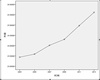time series

• 2020-04-17 09:24:44

# 时间序列预测模型顶会论文资源汇总（2022.04.04更新）

包含时间序列预测，时间序列分类，时间序列异常检测，时空预测，时序推荐等领域的热门模型，以及Transformer应用于时间序列分析的变体和优化。

# 教程汇总与源码获取（2021）

发布该篇博客之后，从去年至今收到了很多私信询问如何入门时间序列分析以及源码，今天终于有时间把过去一年中所学习的知识点和踩过的坑做个梳理，仅供参考，并将总结后的教程和源码，放到GitHub。主要包括以下部分：1）Python；2）TensorFlow/Keras；3）时间序列分析入门教程；4）CNN；5）LSTM；6）时序分类；7）时序预测；8）注意力机制。

Github：https://github.com/datamonday/Time-Series-Analysis-Tutorial，欢迎Star，Fork！持续更新中 ⏰

其它仓库：

1. PythonTricks：Python进阶知识点总结。
2. CPP-Learning：C/C++及CS知识点总结。
3. ROS-Learning：机器人操作系统相关知识总结（Linux + Git + Vim + ROS）。
4. ML-Algorithm-Source-Code：机器学习常用算法源码实现。
5. CV-Learning：计算机视觉相关算法及论文总结。
6. CG-Learning：计算机图形学相关知识总结。
7. NeuroComputing：神经计算与脑机接口相关知识总结。
8. EDA-Beginner：电子设计自动化相关知识总结。
9. FR-AttSys：人脸识别考勤系统设计。
10. MDRL-Notes：机器学习、深度学习、强化学习算法相关知识总结。

# 前言

⏱最一段时间都在学习时间序列预测和时间序列分类相关的知识，一开始拿到任务感觉无从下手，论文看不懂，代码不会写。经过近三个月的摸索，也算是入门的小白了，这篇博文算是个人经过踩坑，去粗取精之后的经验总结。通过4篇博客、9篇论文、32篇实战教程，梳理出了一套系统化的时间序列预测和时间序列分类任务的入门指南。文中提及的博客论文和教程全部内容字数大约在50万字到100万字之间。既是对这段时间所学知识的梳理总结，也希望给有需要的同学提供帮助。水平有限，如有问题欢迎指出，谢谢。

🎯【适合人群】：

• 没有时间序列预测/分类相关的经验的小白

⌨【代码环境】：

• python 3.7.6
• tensorflow 2.1.0

🔊【注意事项】：

• 因为tensorflow 2.0版本开始集成了keras，成为了 tensorflow.keras API，因此不用额外安装，并且无需 import keras

• 本文提及文章的神经网络编写部分使用Keras深度学习框架。

• 本文提及文章的所有代码均在Jupyter Notebook中编写，并测试通过。

• 本文提及的所有文章中，参考资料均在每篇文章的文末给出。

📽【行文顺序】：

• 原理篇：逻辑顺序

• 论文篇：时间顺序

• 实战篇：逻辑顺序+时间顺序

• 注：本文提及的网络架构、论文、教程都是按照由浅入深介绍的，教程部分（三、实战篇）有些教程是有相互关联的。

📖【主要内容】：时间序列任务分为时间序列预测时间序列分类两种类型，本文主要内容如下：

• LSTM 及其不同的网络架构处理时间序列预测/分类任务；
• CNN 及其不同的网络架构处理时间序列预测/分类任务；
• CNN-LSTM 网络处理时间序列预测/分类任务；
• ConvLSTM 网络处理时间序列预测/分类任务；
• DeepConvLSTM 网络处理时间序列分类任务；
• LSTM-FCN 网络处理时间序列分类任务；
• Multivariate LSTM-FCNs 网络处理时间序列分类任务

# 一、原理篇

本部分所有标题都设置了对应文章超链接，直接点击传送。

如果对下文提及的原理比较熟悉，可以直接跳过。

## 1.1 CNN

1. 零基础入门深度学习(4) - 卷积神经网络

• 文章日期：2017/08/28
• 内容梗概：CNN算法原理+数学推导+代码实现

## 1.2 RNN

2. 零基础入门深度学习(5) - 循环神经网络

• 文章日期：2017/08/28
• 内容梗概：RNN算法原理+数学推导+代码实现

## 1.3 LSTM

3. Understanding LSTM Networks

• 文章日期：2015/08/27
• 适合人群：了解LSTM的基本原理。

相信大家看过的很多介绍LSTM原理的文章都用到了这篇文章的配图。比如：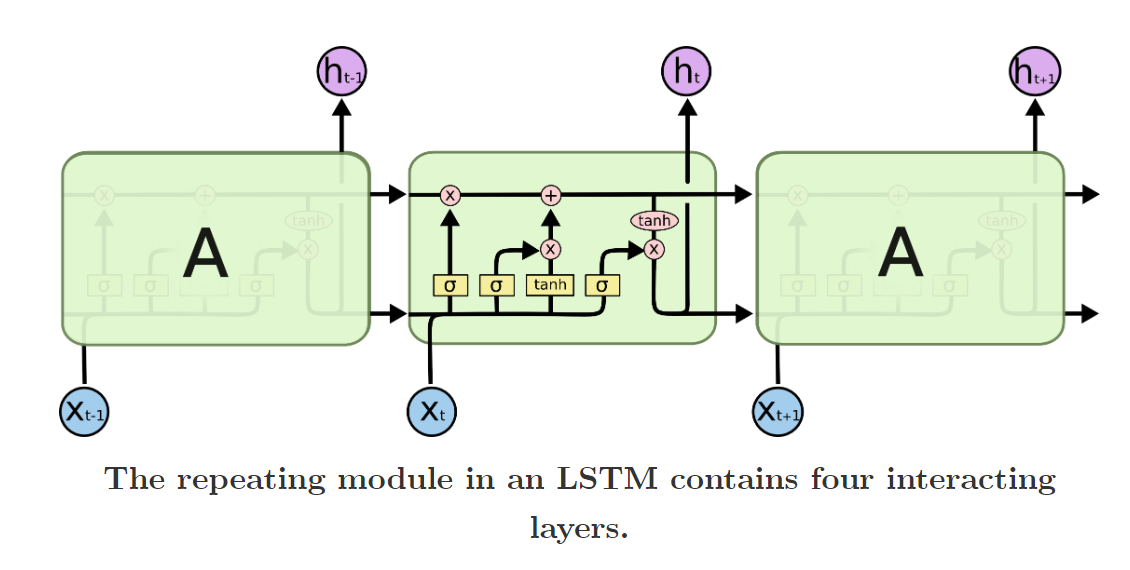4. 零基础入门深度学习(6) - 长短时记忆网络(LSTM)

• 文章日期：2017/08/28
• 内容梗概：LSTM算法原理+数学推导+代码实现

这三篇文章（本篇+之前同系列两篇）应该是我目前遇到的讲解得最详细最全面思路最清晰的文章，由浅入深，原理+推导+代码，墙裂推荐。

# 二、论文篇

本部分主要内容：

• 时间序列分类任务数据集构建（人类活动识别）
• CNN-LSTM 网络
• ConvLSTM 网络
• DeepConvLSTM 网络
• LSTM-FCN 网络

本部分可以先跳过，直接看实战篇，如果对其中的网络架构有疑问或者有些内容看不懂，再阅读这些论文也可以。

## 2.1 WISDM 实验室论文

1. Activity Recognition using Cell Phone Accelerometers

• 论文被引：2034
• 论文年份：2010

WISDM实验室应该是最早开始做基于手机传感器的人类活动识别的实验室，手机的数据只有3个特征（3轴传感器数据）。这篇论文是该实验室的第一篇论文，主要介绍了关于数据集的构建与处理

2. Cell Phone-Based Biometric Identification

• 论文被引：262
• 论文年份：2010

这篇论文是该实验室的第二篇论文，主要介绍了 数据收集以及将时间序列数据转换为样本的过程，值得借鉴。

该实验室还有3篇论文，个人感觉参考意义不大，可以先不看。

## 2.2 UCI-HAR 数据集论文

1. Human Activity Recognition on Smartphones using a Multiclass Hardware-Friendly SVM

• 论文被引：619
• 论文年份：2012

这篇论文是创建该数据集的实验室发表的有关人类活动识别的第一篇论文，主要介绍了使用监督机器学习方法通过手机传感器信号来识别人类活动（6类，分别是站立，行走，放置，行走，上楼和在下楼）进行分类。使用9个特征（6轴传感器+3个分离信号（身体重力加速度））。

2. A Public Domain Dataset for Human Activity Recognition Using Smartphones

• 论文被引：763
• 论文年份：2013

这篇论文介绍了 UCI-HAR Dataset数据集是如何创建的，很有借鉴意义，如果做时间序列分类任务的话，是必读论文

## 2.3 CNN-LSTM 论文

1. Long-term Recurrent Convolutional Networks for Visual Recognition and Description

• 论文被引：3634
• 论文年份：2015

该论文提出的网络架构最初被称为长期循环卷积网络（LRCN），现在使用“CNN LSTM”来指代使用CNN作为前端的LSTM。该网络架构最初用于生成图像的文本描述的任务。关键是CNN，该CNN在图像分类任务上进行了预训练，使得可以用作字幕生成的特征提取器。

2. Show and Tell: A Neural Image Caption Generator

• 论文被引：3378
• 论文年份：2015

该论文提出的网络架构用于语音识别和自然语言处理问题，其中CNN用作音频和文本输入数据上的特征提取器，之后输入到LSTM进行进一步处理。

这两篇论文是CNN-LSTM网络家族的开篇之作，是必读论文。

## 2.4 ConvLSTM 论文

1. Convolutional LSTM Network: A Machine Learning Approach for Precipitation Nowcasting

• 论文被引：1787
• 论文年份：2015

这篇论文是最先提出 ConvLSTM 网络架构的论文，该论文是用于预测相对短时间内某个地区未来的降雨强度，属于必读论文。

## 2.5 DeepConvLSTM 论文

1. Deep Convolutional Neural Networks On Multichannel Time Series For Human Activity Recognition

• 论文被引：541
• 论文年份：2015

在基准数据集上对所提方法与现有方法的比较进行了广泛的研究。结果表明，该方法是一种很有竞争力的HAR问题求解算法。该论文还研究了CNN的效率，并得出结论：CNN对于在线人类活动识别是足够快的

2. Deep Convolutional and LSTM RNN for Multimodal Wearable Activity Recognition

• 论文被引：836
• 论文年份：2016

该论文提出了DeepConvLSTM网络架构：由卷积层和LSTM循环层组成的深度学习框架，它能够自动学习特征表示并对它们的激活之间的时间依赖性进行建模。通过业内的标准的人类活动识别数据集（OPPORTUNITY和Skoda）进行实验，证明此框架适用于可​​穿戴传感器数据的活动识别。属于必读论文。

## 2.6 LSTM-FCN 论文

1. LSTM Fully Convolutional Networks for Time Series Classification

• 论文被引：211
• 论文年份：2017

该论文提出的模型在显著地提高全卷积网络性能的同时保证模型大小只有很少的增加，并且几乎不需要对数据集进行预处理。提出的长短期记忆-全卷积网络 （LSTM-FCN） 与其他网络相比，具有最新的性能。利用注意力-长短期记忆-全卷积网络 （ALSTM-FCN），探讨了注意力机制在时间序列分类中的应用。利用注意力机制可以可视化LSTM细胞的决策过程。此外，还提出了微调的方法来提高训练模型的性能。对模型的性能进行了全面的分析，并与其他技术进行了比较。

2. Multivariate LSTM-FCNs for Time Series Classification

• 论文被引：70
• 论文年份：2018

该论文提出将现有的单变量时间序列分类模型长短期记忆-全卷积网络（LSTM-FCN）和注意力-长短期记忆-全卷积网络（ALSTM-FCN）通过在全卷积块上增加一个压缩和激励块来进一步提高分类精度，从而转化为多变量时间序列分类模型。提出的模型在少量预处理的情况下优于大多数最新模型。所提出的模型能有效地处理各种复杂的多元时间序列分类任务，如活动识别或动作识别。此外，所提出的模型在满足实时要求（小，快），可以部署在内存受限的系统上。

# 三、实战篇

## 3.1 时间序列任务 入门篇

本部分不涉及具体的数据集，使用构造的数字序列；目的是通过程序了解网络架构和定义方法。

# 公众号

欢迎关注公众号，获取更多干货内容！以上是三个多月以来对时间序列预测和分类任务的总结，仅供参考。如果给你带来了帮助，请动动手指点个赞；如果对文中内容存疑，欢迎指出交流，谢谢各位人才。

刚开始的时候，无从下手，走了很多弯路。经过这段时间的试错和摸索，算是总结出了一些经验。上文提到的论文，是个人认为帮助比较大的论文，如果在做时间序列预测或分类任务的话最好看一下，理解其中的建模思路和网络架构的优缺点，以便快速上手。

实战篇的代码主要参考澳大利亚博主Jason Brownlee的博客文章，在此表示感谢。博客地址：传送门tensorflow python
更多相关内容
• python利用支持向量机SVM进行时间序列预测, 包括数据和python代码 python利用支持向量机SVM进行时间序列预测, 包括数据和python代码
• 神经网络 时间序列预测 python语言 code LSTM 深度学习
• tensorflow下用LSTM网络进行时间序列预测，实时多变量预测以及对于未来数据的单变量预测。
• 时间序列预测问题中，建立LSTM模型，采用python语言代码实现
• 时间序列预测讲义（ARIMA&LSTM;）及python代码，首先讲述了基本概念及公式，然后提供了python代码
• ## 时间序列

千次阅读 2018-09-02 00:33:43
时间序列的定义 所谓时间序列就是按照时间的顺序记录的一列有序数据。对时间序列进行观察、研究、找寻他发展变化的规律，预测他将来的走势就是时间序列分析，时间序列分析方法只适用于近期与短期的预测。 相关特征...

# 时间序列的定义

所谓时间序列就是按照时间的顺序记录的一列有序数据。对时间序列进行观察、研究、找寻他发展变化的规律，预测他将来的走势就是时间序列分析，时间序列分析方法只适用于近期与短期的预测。

相关特征统计量：

1. 均值函数序列：反映的是时间序列每时每刻的平均水平

2. 方差函数序列：反映的是时间序列围绕其均值做随机波动时平均的波动程度

3. 协方差函数和相关系数度量的是两个不同的事件彼此之间的相互影响程序。而自协方差函数和自相关系数（ACF）度量的是同一事件在不同时期之间的相关程度，形象地讲就是度量自己过去的行为对自己现在的影响

4. 偏自相关系数：在剔除了中间 k − 1 k-1 个随机变量的干扰后， X t − k 对 X_{t-k}对 X t X_t 影响的相关变量。

时间序列常用模型：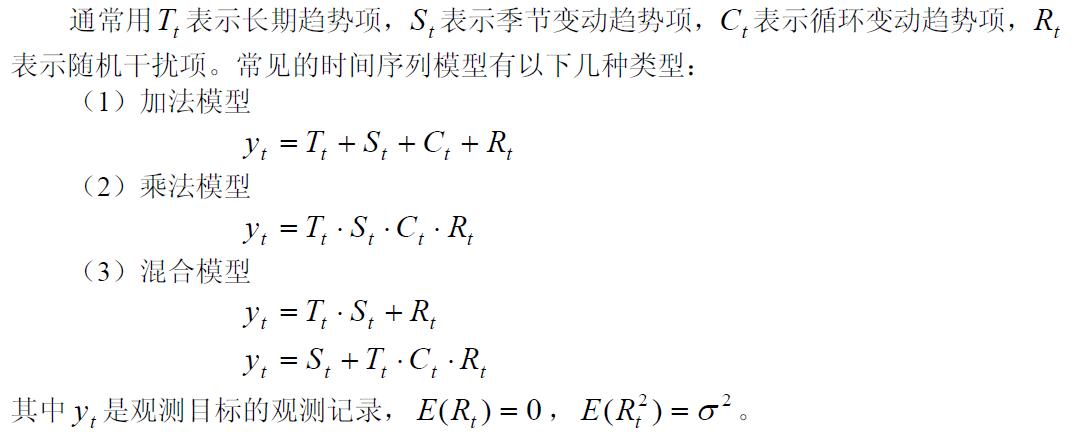• 长期趋势：是时间序列在长时期内呈现出来的持续向上或持续向下的变动。
• 季节变动：是时间序列在一年内重复出现的周期性波动。
• 循环波动：是时间序列呈现出得非固定长度的周期性变动。循环波动的周期可能会持续一段时间，但与趋势不同，它不是朝着单一方向的持续变动，而是涨落相同的交替波动。
• 不规则波动：是时间序列中除去趋势、季节变动和周期波动之后的随机波动。不规则波动通常总是夹杂在时间序列中，致使时间序列产生一种波浪形或震荡式的变动。只含有随机波动的序列也称为平稳序列

# 时间序列分析方法

## 1、移动平均法

缺点：预测值总是停留在过去的水平上而无法预计会导致将来更高或更低的波动

N越大，修匀的程度也越大，起伏也越小，有利于消除不规则变动的影响，但同时周期变动难于反映出来。一般N值的取值范围：5<=N<=200。当历史序列的基本变化不大且序列中随机变动成分较多时，N的取值应该大一些，否则N的取值应该小一些。选择N值的一个有效方法是，比较若干模型的预测误差，预测误差最小者为好。

一次移动平均法

• 使用最近N期序列值的平均值作为未来各期的预测结果，每期数据求平均时的作用是一样的

• 适用范围：适用于发展趋势变化不大的情况

计算公式为：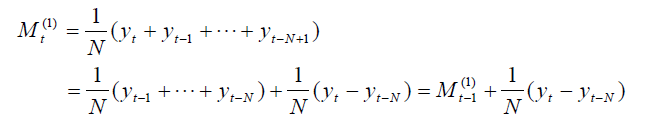预测标准误差为：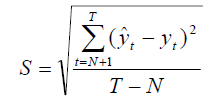加权移动平均法

• 考虑各期数据的重要性，对近期数据给予较大的权重。

• 需要计算总的平均相对误差，修正预测值

• 适用范围：适用于发展趋势变化不大的情况

计算公式为：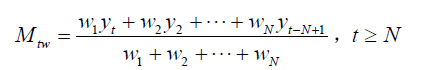二次移动平均法

• 利用移动平均的滞后偏差的规律来建立直线趋势的预测模型，是一种既能反映趋势变化，又可以有效分离出周期变化的方法。

• 适用范围：适用于具有线性趋势的情况

计算公式为：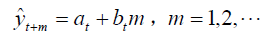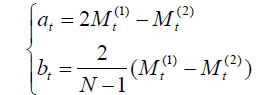####2、指数平滑法####

指数平滑法实际上是一种特殊的加权移动平均法。预测值是以前观测值的加权和，且对不同的数据给予不同的权，新数据给较大的权，旧数据给较小的权。

缺点：难以确定指数平滑系数，受主观影响较大

一次指数平滑法

• 适用范围：适用于发展趋势变化不大的情况

计算公式为：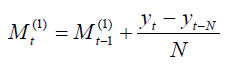即：最新预测值=前一期预测值+前期预测值产生的误差的修正值。

平滑系数的确定：

• 平滑系数a起到一个调节器的作用。a值选取得越大，预测值受近期影响越大；a值选取得越小，预测值受远期影响越大。
• 如果时间序列波动不大，比较平稳，则a应取小一点（如0.1~0.5），以减少修正幅度，使预测模型能包含较长时间序列的信息
• 如果时间序列具有迅速且明显的变动倾向，则a应取大一点，如（0.6~0.8）

初始值的确定：

• 当时间序列的数据较多时，取第一期的实际值为初值
• 当时间序列的数据较少时，取最初几期的平均值为初值

二次指数平滑法

• 适用范围：适用于具有线性趋势的情况

计算公式为：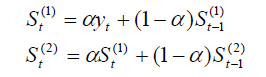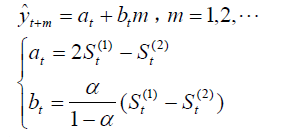# 平稳时间序列

一个时间序列，如果均值没有系统的变化（无趋势）、方差没有系统变化，且严格消除了周期性变化，就称之是平稳的。

根据限制条件的严格程度，平稳时间序列可以分为以下两种类型

• 严平稳时间序列：只有当序列的所有统计特征都不会随时间而变化时才能被称为严平稳。是对序列联合分布的要求，以保证序列所有的统计特征都相同

• 宽平稳时间序列：只要求序列二阶平稳，对于高于二阶的维度没有任何要求

平稳时间序列有以下两个统计性质

• 常数均值

• 自协方差函数和自相关函数只依赖于时间的平移长度而与时间的起止点无关

# 时间序列的预处理

对序列进行纯随机值和平稳性的检验

## 1、平稳性的检验

对序列的平稳性有两种检验方法，一种是根据时序图和自相关图显示的特征做出判断的图检验方法；一种是构造检验统计量进行假设检验的方法。图检验方法操作简便，但其主观性较强，最好能用假设检验的方法进行辅助判断。

时序图检验

• 根据平稳时间序列方差和均值为常数的特点，平稳序列的时序图应该显示出该序列始终在一个常数值附近随机波动，并且波动的范围由界的特点。

• 如果观察序列的时序图显示出该序列有明显的趋势性或周期性，那它通常不是平稳序列。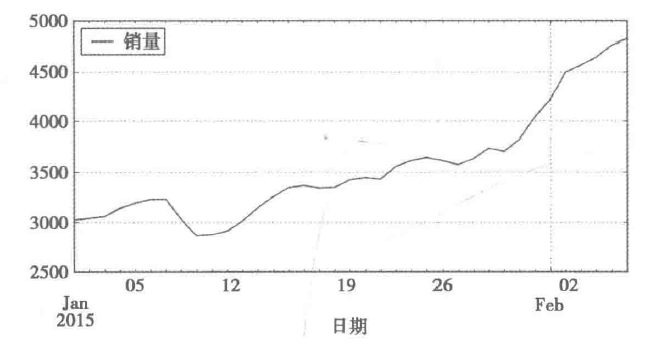如图所示，销量有明显的上升趋势，所以它一定不是平稳序列。

自相关图检验

• 自相关图的一个坐标轴表示延迟时间数，另一个坐标轴表示自相关系数

• 平稳序列通常具有短期自相关性：随着延迟期数的增加，平稳序列的自相关性会很快衰减到0。反之，非平稳序列的自相关系数衰减向0的速度会比较慢。

三角对称性：具有单调趋势的非平稳序列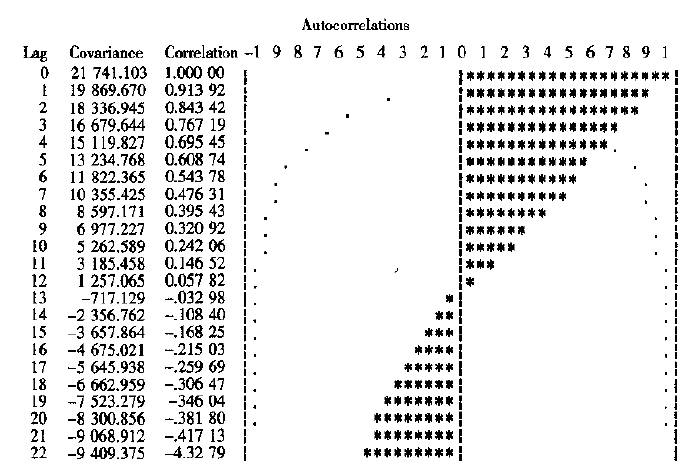长期位于零轴的一边：具有单调趋势的非平稳序列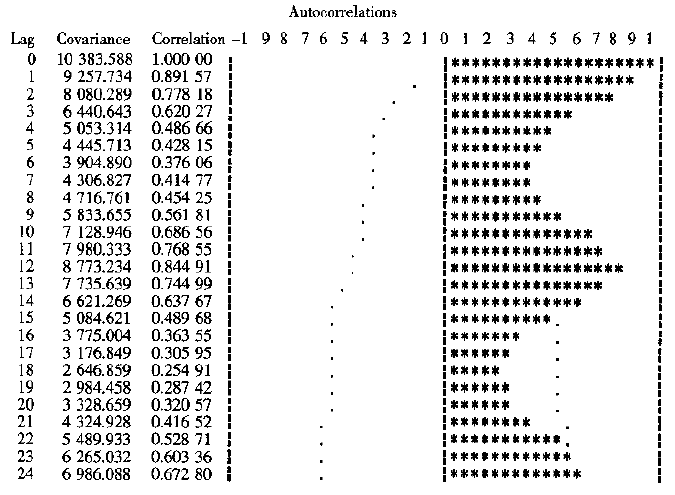正弦波动规律：具有周期变化规律的非平稳序列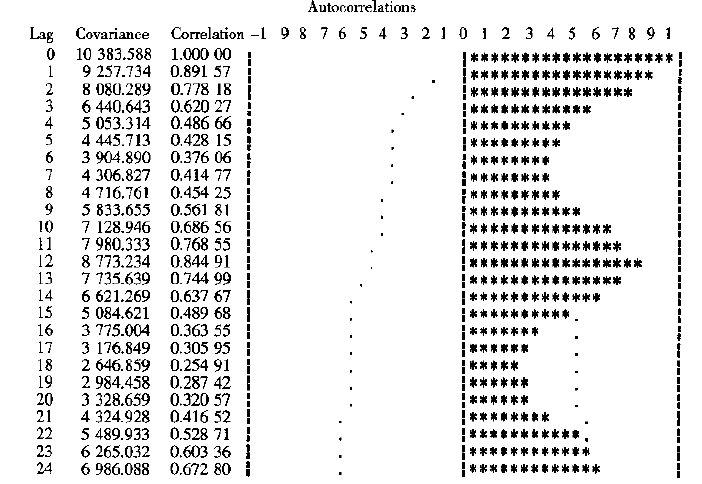在零轴附近波动：随机性强的平稳时间序列（很快衰减到0）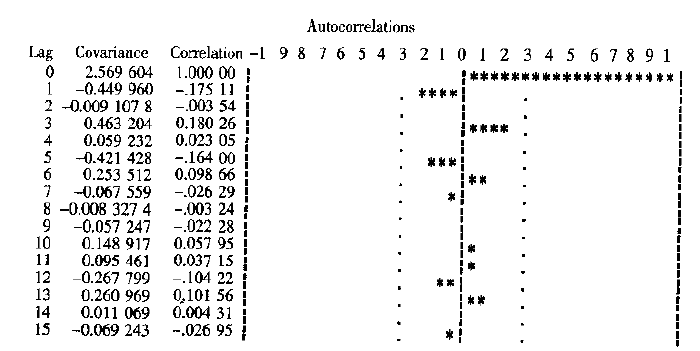单位根检验

如果该统计量的P值小于a时，则可以以1-a的置信水平拒绝原假设，认为该序列为平稳序列；否则，接受原假设，认为该序列为非平稳序列。假设a为0.05，此时p值显著大于0.05，则该序列为非平稳序列。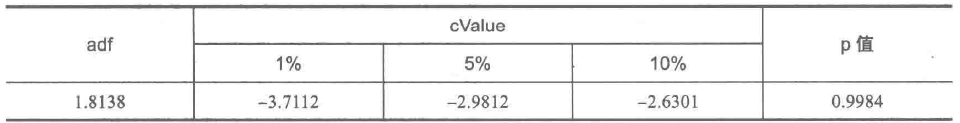## 2、纯随机性的检验

纯随机序列又称为白噪声序列。如果序列值之间没有任何的相关性，这种序列我们称为白噪声序列。白噪声序列没有任何分析的价值

• 非平稳序列一定不是白噪声序列
• 平稳序列如果显示出显著的短期相关性，那么该序列一定不是白噪声序列，否则要对其进行纯随机性检验

如果该统计量的P值小于a时，则可以以1-a的置信水平拒绝原假设，认为该序列为非白噪声序列；否则，接受原假设，认为该序列为纯随机序列。假设a为0.05，此时p值显著小于0.05，则该序列为非白噪声序列。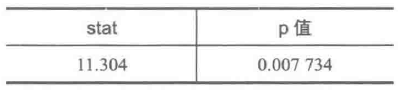# 平稳时间序列分析

某个时间序列经过预处理，被判定为平稳非白噪声序列，就可以利用ARMA模型进行建模

建模步骤：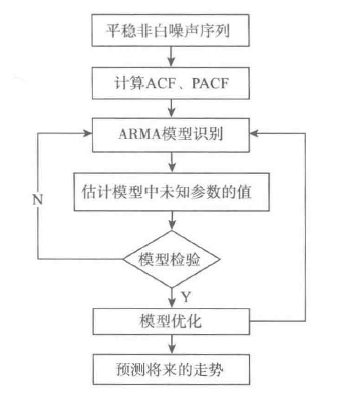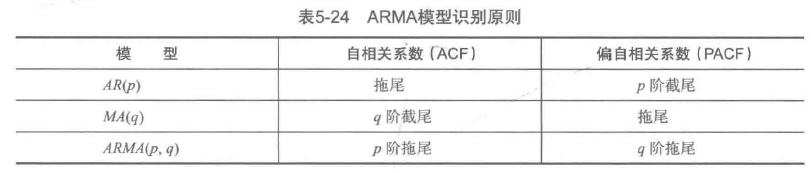# 非平稳时间序列分析

ARIMA模型的实质就是差分运算与ARMA模型的组合。

差分运算：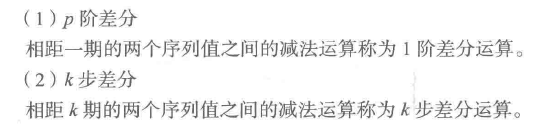建模步骤：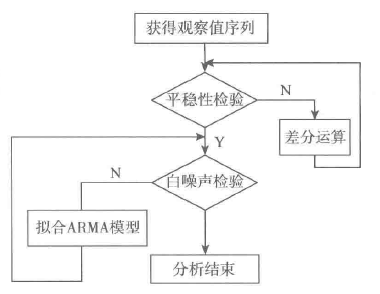展开全文• 用 LSTM 做时间序列预测的一个小例子，详情见我滴博文。
• 时间序列分析及应用:R语言 原书第2版 》以易于理解的方式讲述了时间序列模型及其应用 主要内容包括：趋势 平稳时间序列模型 非平稳时间序列模型 模型识别 参数估计 模型诊断 预测 季节模型 时间序列回归模型 异...
• 时间序列预测建模，移动平滑、指数平滑、等模型的描述讲解和matlab程序实现代码。arima、arma等等
• 时间序列定义： 时间序列是按照一定的时间间隔排列的一组数据，其时间间隔可以是任意的时间单位，如小时、日、周月等。比如，每天某产品的用户数量，每个月的销售额，这些数据形成了以一定时间间隔的数据。 通过对...

# 时间序列系列文章：

时间序列及其预测是日常工作中建模，分析，预测的重要组成部分。本系列我们将从0开始介绍时间序列的含义，模型及其分析。本篇为第一部分，我们主要介绍时间序列，与其常用的预测模型。

# 时间序列定义：

时间序列是按照一定的时间间隔排列的一组数据，其时间间隔可以是任意的时间单位，如小时、日、周月等。比如，每天某产品的用户数量，每个月的销售额，这些数据形成了以一定时间间隔的数据。

通过对这些时间序列的分析，从中发现和揭示现象发展变化的规律，并将这些知识和信息用于预测。比如销售量是上升还是下降，销售量是否与季节有关，是否可以通过现有的数据预测未来一年的销售额是多少等。

对于时间序列的预测，由于很难确定它与其他变量之间的关系，这时我们就不能用回归去预测，而应使用时间序列方法进行预测。

采用时间序列分析进行预测时需要一系列的模型，这种模型称为时间序列模型。

# 时间序列预测模型与方法

注：本部分只关注相关模型与分析的方法，模型的选择，调参与优化会放在后续文章中详细讲解

## 原始数据

本文所使用原始数据与代码，可以在公众号：Smilecoc的杂货铺 中回复“时间序列”获取。可直接扫描文末二维码关注！

## 朴素法

朴素法就是预测值等于实际观察到的最后一个值。它假设数据是平稳且没有趋势性与季节性的。通俗来说就是以后的预测值都等于最后的值。

这种方法很明显适用情况极少，所以我们重点通过这个方法来熟悉一下数据可视化与模型的评价及其相关代码。

#朴素法
dd = np.asarray(train['Count'])#训练组数据
y_hat = test.copy()#测试组数据
y_hat['naive'] = dd[len(dd) - 1]#预测组数据

#数据可视化
plt.figure(figsize=(12, 8))
plt.plot(train.index, train['Count'], label='Train')
plt.plot(test.index, test['Count'], label='Test')
plt.plot(y_hat.index, y_hat['naive'], label='Naive Forecast')
plt.legend(loc='best')
plt.title("Naive Forecast")
plt.show()


得到结果：我们通过计算均方根误差，检查模型在测试数据集上的准确率。
其中均方根误差（RMSE）是各数据偏离真实值的距离平方和的平均数的开方

#计算均方根误差RMSE
from sklearn.metrics import mean_squared_error
from math import sqrt

# mean_squared_error求均方误差
rmse = sqrt(mean_squared_error(test['Count'], y_hat['naive']))
print(rmse)


得到均方根误差为1053

## 简单平均法

简单平均法就是预测的值为之前过去所有值的平均.当然这不会很准确，但这种预测方法在某些情况下效果是最好的。

#简单平均法
y_hat_avg = test.copy()
y_hat_avg['avg_forecast'] = train['Count'].mean()


其后续可视化与模型效果评估方法与上述一致，这里不再赘述，需要详细代码可以查看相关源码。得到RMSE值为2637

## 移动平均法

我们经常会遇到这种数据集，比如价格或销售额某段时间大幅上升或下降。如果我们这时用之前的简单平均法，就得使用所有先前数据的平均值，但在这里使用之前的所有数据是说不通的，因为用开始阶段的价格值会大幅影响接下来日期的预测值。因此，我们只取最近几个时期的价格平均值。很明显这里的逻辑是只有最近的值最要紧。这种用某些窗口期计算平均值的预测方法就叫移动平均法。

#移动平均法
y_hat_avg = test.copy()
#利用时间窗函数rolling求平均值u
y_hat_avg['moving_avg_forecast'] = train['Count'].rolling(60).mean().iloc[-1]


其后续可视化与模型效果评估方法与上述一致，这里不再赘述，需要详细代码可以查看相关源码。得到RMSE值为1121

## 指数平滑法

在做时序预测时，一个显然的思路是：认为离着预测点越近的点，作用越大。比如我这个月体重100斤，去年某个月120斤，显然对于预测下个月体重而言，这个月的数据影响力更大些。假设随着时间变化权重以指数方式下降——最近为0.8，然后0.8**2，0.8**3…，最终年代久远的数据权重将接近于0。将权重按照指数级进行衰减，这就是指数平滑法的基本思想。

指数平滑法有几种不同形式：一次指数平滑法针对没有趋势和季节性的序列，二次指数平滑法针对有趋势但没有季节性的序列，三次指数平滑法针对有趋势也有季节性的序列。“

所有的指数平滑法都要更新上一时间步长的计算结果，并使用当前时间步长的数据中包含的新信息。它们通过”混合“新信息和旧信息来实现，而相关的新旧信息的权重由一个可调整的参数来控制。

### 一次指数平滑

一次指数平滑法的递推关系如下：

s i = α x i + ( 1 − α ) s i − 1 ， 其 中 0 ≤ α ≤ 1 s_{i}=\alpha x_{i}+(1-\alpha)s_{i-1}，其中 0 \leq \alpha \leq 1

其中， s i s_{i} 是时间步长i（理解为第i个时间点）上经过平滑后的值， x i x_{i} 是这个时间步长上的实际数据。 α \alpha 可以是0和1之间的任意值，它控制着新旧信息之间的平衡：当 α \alpha 接近1，就只保留当前数据点；当 α \alpha 接近0时，就只保留前面的平滑值(整个曲线都是平的)。我们展开它的递推关系式：

我们展开它的递推关系式：
s i = α x i + ( 1 − α ) s i − 1 = α x i + ( 1 − α ) [ α x i − 1 + ( 1 − α ) s i − 2 ] = α x i + ( 1 − α ) [ α x i − 1 + ( 1 − α ) [ α x i − 2 + ( 1 − α ) s i − 3 ] ] = α [ x i + ( 1 − α ) x i − 1 + ( 1 − α ) 2 x i − 2 + ( 1 − α ) 3 s i − 3 ] = . . . = α ∑ j = 0 i ( 1 − α ) j x i − j \begin{aligned} s_{i}&=\alpha x_{i}+(1-\alpha)s_{i-1} \\ &=\alpha x_{i}+(1-\alpha)[\alpha x_{i-1}+(1-\alpha)s_{i-2}]\\ &=\alpha x_{i}+(1-\alpha)[\alpha x_{i-1}+(1-\alpha)[\alpha x_{i-2}+(1-\alpha)s_{i-3}]]\\ &=\alpha[x_{i}+(1-\alpha)x_{i-1}+(1-\alpha)^{2}x_{i-2}+(1-\alpha)^{3}s_{i-3}]\\ &=... \\ &=\alpha\sum_{j=0}^{i}(1-\alpha)^{j}x_{i-j} \end{aligned}

可以看出，在指数平滑法中，所有先前的观测值都对当前的平滑值产生了影响，但它们所起的作用随着参数 α \alpha 的幂的增大而逐渐减小。那些相对较早的观测值所起的作用相对较小。同时，称α为记忆衰减因子可能更合适——因为α的值越大，模型对历史数据“遗忘”的就越快。从某种程度来说，指数平滑法就像是拥有无限记忆（平滑窗口足够大）且权值呈指数级递减的移动平均法。一次指数平滑所得的计算结果可以在数据集及范围之外进行扩展，因此也就可以用来进行预测。预测方式为：

x i + h = s i x_{i+h}=s_{i}

s i s_{i} 是最后一个已经算出来的值。h等于1代表预测的下一个值。

我们可以通过statsmodels中的时间序列模型进行指数平滑建模。官方文档地址为：
https://www.statsmodels.org/stable/generated/statsmodels.tsa.holtwinters.SimpleExpSmoothing.html
具体代码如下：

#一次指数平滑
from statsmodels.tsa.api import SimpleExpSmoothing

y_hat_avg = test.copy()
fit = SimpleExpSmoothing(np.asarray(train['Count'])).fit(smoothing_level=0.6, optimized=False)
y_hat_avg['SES'] = fit.forecast(len(test))


之后同样进行数据可视化并查看模型效果

plt.figure(figsize=(16, 8))
plt.plot(train['Count'], label='Train')
plt.plot(test['Count'], label='Test')
plt.plot(y_hat_avg['SES'], label='SES')
plt.legend(loc='best')
plt.show()


可视化结果为：RMSE结果为1040

### 二次指数平滑

在介绍二次指数平滑前介绍一下趋势的概念。

趋势，或者说斜率的定义很简单： b = Δ y / Δ x b=Δy/Δx ，其中 Δ x Δx 为两点在x坐标轴的变化值，所以对于一个序列而言，相邻两个点的 Δ x = 1 Δx=1 ，因此 b = Δ y = y ( x ) − y ( x − 1 ) b=Δy=y(x)-y(x-1) 。 除了用点的增长量表示，也可以用二者的比值表示趋势。比如可以说一个物品比另一个贵20块钱，等价地也可以说贵了5%，前者称为可加的（addtive），后者称为可乘的（multiplicative）。在实际应用中，可乘的模型预测稳定性更佳，但是为了便于理解，我们在这以可加的模型为例进行推导。
指数平滑考虑的是数据的baseline，二次指数平滑在此基础上将趋势作为一个额外考量，保留了趋势的详细信息。即我们保留并更新两个量的状态：平滑后的信号和平滑后的趋势。公式如下：
基准等式
s i = α x i + ( 1 − α ) ( s i − 1 + t i − 1 ) s_{i}=\alpha x_{i}+(1-\alpha)(s_{i-1}+t_{i-1})
趋势等式
t i = β ( s i − s i − 1 ) + ( 1 − β ) t i − 1 t_{i}=\beta (s_{i}-s_{i-1})+(1-\beta)t_{i-1}

第二个等式描述了平滑后的趋势。当前趋势的未平滑“值”（ t i t_{i} ）是当前平滑值（ s i s_{i} ）和上一个平滑值（ s i − 1 s_{i-1} ）的差；也就是说，当前趋势告诉我们在上一个时间步长里平滑信号改变了多少。要想使趋势平滑，我们用一次指数平滑法对趋势进行处理，并使用参数 β \beta （理解：对 t i t_{i} 的处理类似于一次平滑指数法中的 s i s_{i} ，即对趋势也需要做一个平滑，临近的趋势权重大）。

为获得平滑信号，我们像上次那样进行一次混合，但要同时考虑到上一个平滑信号及趋势。假设单个步长时间内保持着上一个趋势，那么第一个等式的最后那项就可以对当前平滑信号进行估计。

若要利用该计算结果进行预测，就取最后那个平滑值，然后每增加一个时间步长就在该平滑值上增加一次最后那个平滑趋势：

x i + h = s i + h t i x_{i+h}=s_{i}+ht_{i}之后使用二次指数平滑进行预测：

from statsmodels.tsa.api import Holt

y_hat_avg = test.copy()

fit = Holt(np.asarray(train['Count'])).fit(smoothing_level=0.3, smoothing_slope=0.1)
y_hat_avg['Holt_linear'] = fit.forecast(len(test))


结果如图：得到对应的RMSE为1033

### 三次指数平滑

在应用这种算法前，我们先介绍一个新术语。假如有家酒店坐落在半山腰上，夏季的时候生意很好，顾客很多，但每年其余时间顾客很少。因此，每年夏季的收入会远高于其它季节，而且每年都是这样，那么这种重复现象叫做“季节性”（Seasonality）。如果数据集在一定时间段内的固定区间内呈现相似的模式，那么该数据集就具有季节性。二次指数平滑考虑了序列的基数和趋势，三次就是在此基础上增加了一个季节分量。类似于趋势分量，对季节分量也要做指数平滑。比如预测下一个季节第3个点的季节分量时，需要指数平滑地考虑当前季节第3个点的季节分量、上个季节第3个点的季节分量…等等。详细的有下述公式(累加法)：

s i = α ( x i − p i − k ) + ( 1 − α ) ( s i − 1 + t i − 1 ) t i = β ( s i − s i − 1 ) + ( 1 − β ) t i − 1 p i = γ ( x i − s i ) + ( 1 − γ ) p i − k \begin{aligned} s_{i}&=\alpha (x_{i}-p_{i-k})+(1-\alpha)(s_{i-1}+t_{i-1}) \\ t_{i} &=\beta (s_{i}-s_{i-1})+(1-\beta)t_{i-1}\\ p_{i}&=\gamma (x_{i}-s_{i})+(1-\gamma)p_{i-k} \end{aligned}

其中， p i p_{i} 是指“周期性”部分。预测公式如下：

x i + h = s i + h t i + p i − k + h x_{i+h}=s_{i}+ht_{i}+p_{i-k+h}

k 是这个周期的长度。

在使用二次平滑模型与三次平滑模型前，我们可以使用sm.tsa.seasonal_decompose分解时间序列，可以得到以下分解图形——从上到下依次是原始数据、趋势数据、周期性数据、随机变量（残差值）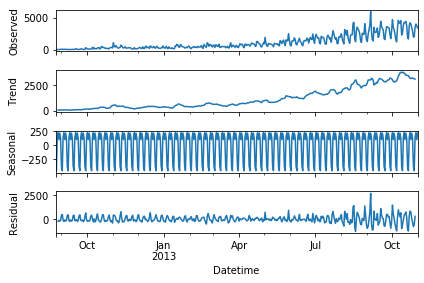根据分析图形和数据可以确定对应的季节参数

具体代码为：

#三次指数平滑
from statsmodels.tsa.api import ExponentialSmoothing

y_hat_avg = test.copy()
y_hat_avg['Holt_Winter'] = fit1.forecast(len(test))得到的RMSE为575。我们可以看到趋势和季节性的预测准确度都很高。你可以试着调整参数来优化这个模型。

# AR模型

AR(Auto Regressive Model)自回归模型是线性时间序列分析模型中最简单的模型。通过自身前面部分的数据与后面部分的数据之间的相关关系（自相关）来建立回归方程，从而可以进行预测或者分析。服从p阶的自回归方程表达式如下：

x t = ϕ 1 x t − 1 + ϕ 2 x t − 2 + ⋯ + ϕ p x t − p + μ t x_{t}=\phi_{1}x_{t-1}+\phi_{2}x_{t-2}+\cdots+\phi_{p}x_{t-p}+\mu_{t}

表示为 A R ( p ) AR(p) ,。其中， μ t \mu_{t} 表示白噪声，是时间序列中的数值的随机波动，但是这些波动会相互抵消，最终是0。 ϕ \phi 表示自回归系数。

所以当只有一个时间记录点时，称为一阶自回归过程，即AR(1)。其表达式为：
x t = ϕ 1 x t − 1 + μ t x_{t}=\phi_{1}x_{t-1}+\mu_{t}

利用Python建立AR模型一般会用到我们之后会说到的ARIMA模型（AR模型中的p是ARIMA模型中的参数之一，只要将其他的参数设置为0即为AR模型）。您可以先阅读后续ARIMA模型的内容并参考文件中的代码查看具体的内容

# MA模型

MA(Moving Average Model)移动平均模型通过将一段时间序列中白噪声(误差)进行加权和，可以得到移动平均方程。如下模型为q阶移动平均过程，表示为MA(q)。

x t = μ + μ t + θ 1 μ t − 1 + θ 2 μ t − 2 + ⋯ + θ q μ t − q x_{t}=\mu+\mu_{t}+\theta_{1}\mu_{t-1}+\theta_{2}\mu_{t-2}+\cdots+\theta_{q}\mu_{t-q}

其中 x t x_{t} 表示t期的值，当期的值由前q期的误差值来决定， μ μ 值是常数项，相当于普通回归中的截距项， μ t \mu_{t} 是当期的随机误差。MA模型的核心思想是每一期的随机误差都会影响当期值，把前q期的所有误差加起来就是对t期值的影响。

同样，利用Python建立MA模型一般会用到我们之后会说到的ARIMA模型,您可以先阅读后续ARIMA模型的内容并参考文件中的代码查看具体的内容

# ARMA模型

ARMA(Auto Regressive and Moving Average Model)自回归移动平均模型是与自回归和移动平均模型两部分组成。所以可以表示为ARMA(p, q)。p是自回归阶数，q是移动平均阶数。

x t = ϕ 1 x t − 1 + ϕ 2 x t − 2 + ⋯ + ϕ p x t − p + μ t + θ 1 μ t − 1 + θ 2 μ t − 2 + ⋯ + θ q μ t − q x_{t}=\phi_{1}x_{t-1}+\phi_{2}x_{t-2}+\cdots+\phi_{p}x_{t-p}+\mu_{t}+\theta_{1}\mu_{t-1}+\theta_{2}\mu_{t-2}+\cdots+\theta_{q}\mu_{t-q}

从式子中就可以看出，自回归模型结合了两个模型的特点，其中，AR可以解决当前数据与后期数据之间的关系，MA则可以解决随机变动也就是噪声的问题。

# ARIMA模型

ARIMA(Auto Regressive Integrate Moving Average Model)差分自回归移动平均模型是在ARMA模型的基础上进行改造的，ARMA模型是针对t期值进行建模的，而ARIMA是针对t期与t-d期之间差值进行建模，我们把这种不同期之间做差称为差分，这里的d是几就是几阶差分。ARIMA模型也是基于平稳的时间序列的或者差分化后是稳定的，另外前面的几种模型都可以看作ARIMA的某种特殊形式。表示为ARIMA(p, d, q)。p为自回归阶数，q为移动平均阶数，d为时间成为平稳时所做的差分次数，也就是Integrate单词的在这里的意思。

具体步骤如下：

x t = ϕ 1 w t − 1 + ϕ 2 w t − 2 + ⋯ + ϕ p w t − p + μ t + θ 1 μ t − 1 + θ 2 μ t − 2 + ⋯ + θ q μ t − q x_{t}=\phi_{1}w_{t-1}+\phi_{2}w_{t-2}+\cdots+\phi_{p}w_{t-p}+\mu_{t}+\theta_{1}\mu_{t-1}+\theta_{2}\mu_{t-2}+\cdots+\theta_{q}\mu_{t-q}

上面公式中的 w t w_{t} 表示t期经过d阶差分以后的结果。我们可以看到ARIMA模型的形式基本与ARMA的形式是一致的，只不过把 X X 换成了 w w

使用ARIMA进行预测代码如下：

from statsmodels.tsa.arima_model import ARIMA

ts_ARIMA= train['Count'].astype(float)
fit1 = ARIMA(ts_ARIMA, order=(7, 1, 4)).fit()
y_hat_ARIMA = fit1.predict(start="2013-11-1", end="2013-12-31", dynamic=True)


并画出预测值与实际值图形：

plt.figure(figsize=(16, 8))
plt.plot(train['Count'], label='Train')
plt.plot(test['Count'], label='Test')
plt.plot(y_hat_ARIMA, label='ARIMA')
plt.legend(loc='best')
plt.show()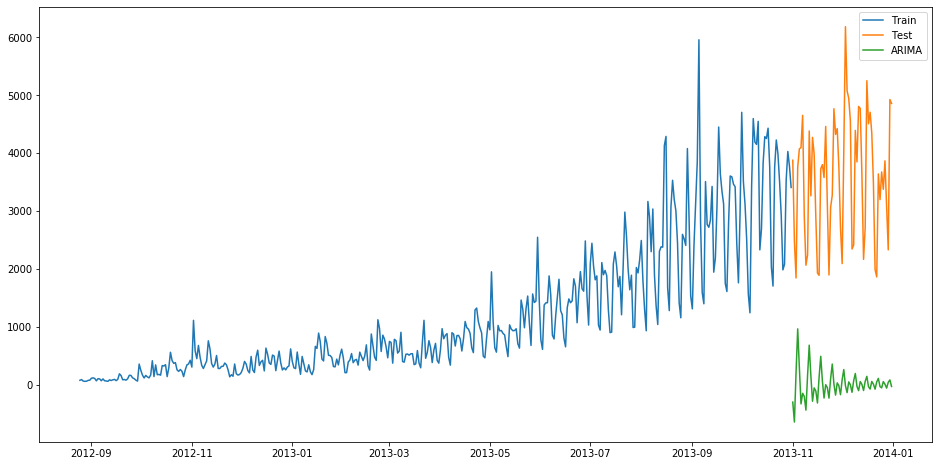并计算RMSE:

from sklearn.metrics import mean_squared_error
from math import sqrt

rmse = sqrt(mean_squared_error(test['Count'],y_hat_ARIMA.to_frame()))
print(rmse)


得到对应的RMSE为3723

# SARIMA模型

SARIMA季节性自回归移动平均模型模型在ARIMA模型的基础上添加了季节性的影响，结构参数有七个：SARIMA(p,d,q)(P,D,Q,s)
其中p,d,q分别为之前ARIMA模型中我们所说的p:趋势的自回归阶数。d:趋势差分阶数。q:趋势的移动平均阶数。
P:季节性自回归阶数。
D:季节性差分阶数。
Q:季节性移动平均阶数。
s:单个季节性周期的时间步长数。

import statsmodels.api as sm
y_hat_avg = test.copy()
fit1 = sm.tsa.statespace.SARIMAX(train.Count, order=(2, 1, 4), seasonal_order=(0, 1, 1, 7)).fit()
y_hat_avg['SARIMA'] = fit1.predict(start="2013-11-1", end="2013-12-31", dynamic=True)


得到实际值与预测值如下：

plt.figure(figsize=(16, 8))
plt.plot(train['Count'], label='Train')
plt.plot(test['Count'], label='Test')
plt.plot(y_hat_avg['SARIMA'], label='SARIMA')
plt.legend(loc='best')
plt.show()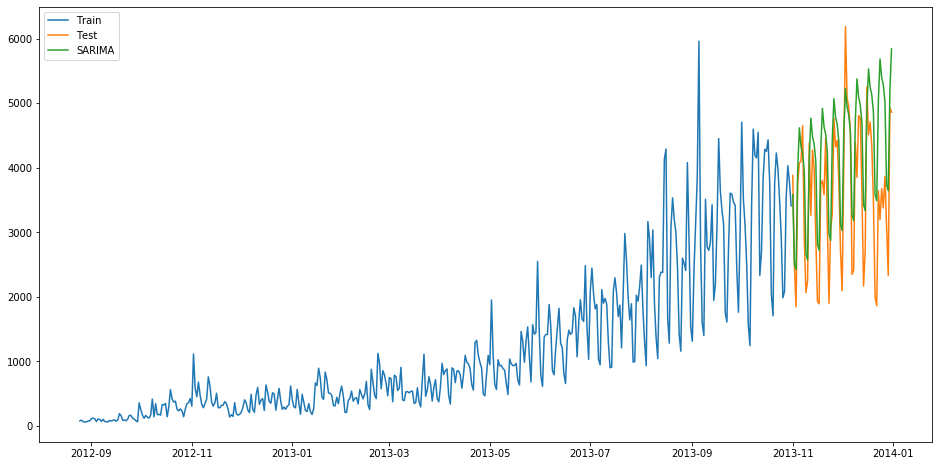并计算RMSE：

from sklearn.metrics import mean_squared_error
from math import sqrt
rmse = sqrt(mean_squared_error(test['Count'], y_hat_avg['SARIMA']))
print(rmse)


结果为933

其他时间序列预测的模型还有SARIMAX模型（在ARIMA模型上加了季节性的因素），Prophet模型，ARCH模型，LSTM神经网络模型等。限于篇幅，感兴趣的同学可以自行查看相关模型资料

在后续的文章中我们将讲解如何确定数据的平稳性与数据预处理，为后续时间序列的建模做准备

相关代码与数据可关注公众号并回复：时间序列获取展开全文python
• Datawhale干货译者：陈超，北京大学，数据派THU 本文约7500字，建议阅读20+分钟本文介绍了时间序列的定义、特征并结合实例给出了时间序列在Python中评价指标和方法。...

Datawhale干货

译者：陈超北京大学，数据派THU



本文约7500字，建议阅读20+分钟本文介绍了时间序列的定义、特征并结合实例给出了时间序列在Python中评价指标和方法。


时间序列是在规律性时间间隔上记录的观测值序列。本指南将带你了解在Python中分析给定时间序列的特征的全过程。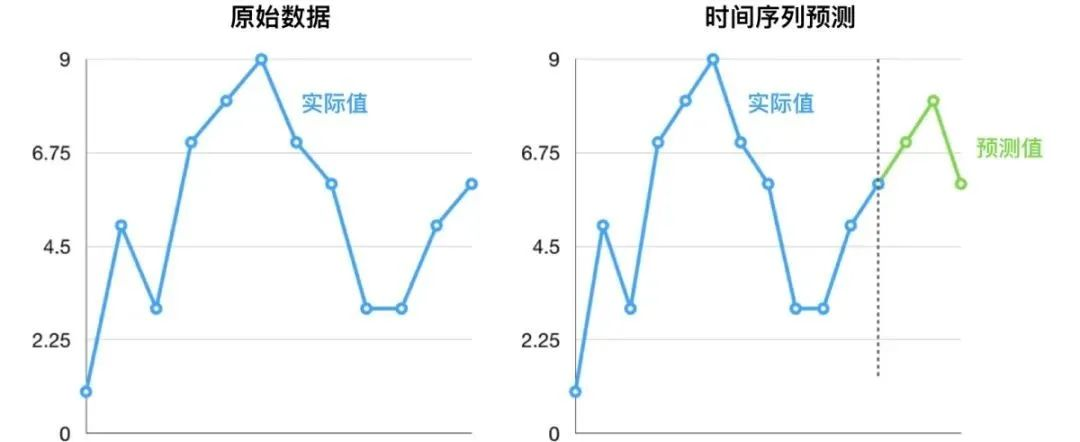主要内容

1. 什么是时间序列？

2. 如何在Python中导入时间序列？

3. 什么是面板数据？

4. 时间序列可视化

5. 时间序列的模式

6. 时间序列的加法和乘法

7. 如何将时间序列分解？

8. 平稳和非平稳时间序列

9. 如何获取平稳的时间序列？

10. 如何检验平稳性？

11. 白噪音和平稳序列的差异是什么？

12. 如何去除时间序列的线性分量？

13. 如何消除时间序列的季节性？

14. 如何检验时间序列的季节性？

15. 如何处理时间序列中的缺失值？

16. 什么是自回归和偏自回归函数？

17. 如何计算偏自回归函数？

18. 滞后图

19. 如何估计时间序列的预测能力？

20. 为什么以及怎样使时间序列平滑？

21. 如何使用Granger因果检验来获知时间序列是否对预测另一个序列帮助？

22. 下一步是什么?

1. 什么是时间序列?

时间序列是在规律性时间间隔记录的观测值序列。

依赖于观测值的频率，典型的时间序列可分为每小时、每天、每周、每月、每季度和每年为单位记录。有时，你可能也会用到以秒或者分钟为单位的时间序列，比如，每分钟用户点击量和访问量等等。

1.1 为什么要分析时间序列呢？

因为它是你做序列预测前的一步准备过程。而且，时间序列预测拥有巨大的商业重要性，因为对商业来说非常重要的需求和销量、网站访问人数、股价等都是时间序列数据。

1.2 所以时间序列分析包括什么内容呢？

时间序列分析包括理解序列内在本质的多个方面以便于你可更好地了解如何做出有意义并且精确的预测。

## 2. 如何在Python中导入时间序列？

所以怎样导入时间序列数据呢？典型的时间序列数据以.csv格式或者其他表格形式存储，包括两列：日期和测量值。

from dateutil.parser import parse
import matplotlib as mpl
import matplotlib.pyplot as plt
import seaborn as sns
import numpy as np
import pandas as pd
plt.rcParams.update({'figure.figsize': (10, 7), 'figure.dpi': 120})

# Import as Dataframe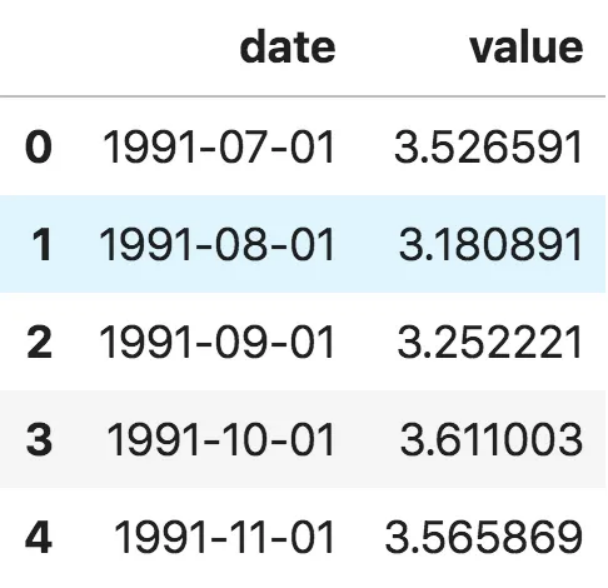数据框时间序列

ser = pd.read_csv('https://raw.githubusercontent.com/selva86/datasets/master/a10.csv', parse_dates=['date'], index_col='date')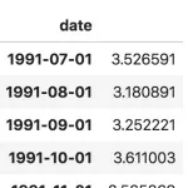时间序列

注意，在此序列当中，‘value’列的位置高于date以表明它是一个序列。

3. 什么是面板数据？

面板数据也是基于时间的数据集。

差异在于，除了时间序列，它也包括同时测量的一个或多个相关变量。

通常来看，面板数据当中的列包括了有助于预测Y的解释型变量，假设这些列将在未来预测阶段有用。

面板数据的例子如下：

# dataset source: https://github.com/rouseguy
df = df.loc[df.market=='MUMBAI', :]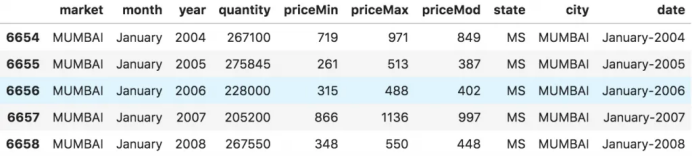面板数据

## 4. 时间序列可视化

让我们用matplotlib来对序列进行可视化。

# Time series data source: fpp pacakge in R.
import matplotlib.pyplot as plt

# Draw Plot
def plot_df(df, x, y, title="", xlabel='Date', ylabel='Value', dpi=100):
plt.figure(figsize=(16,5), dpi=dpi)
plt.plot(x, y, color='tab:red')
plt.gca().set(title=title, xlabel=xlabel, ylabel=ylabel)
plt.show()

plot_df(df, x=df.index, y=df.value, title='Monthly anti-diabetic drug sales in  Australia from 1992 to 2008.')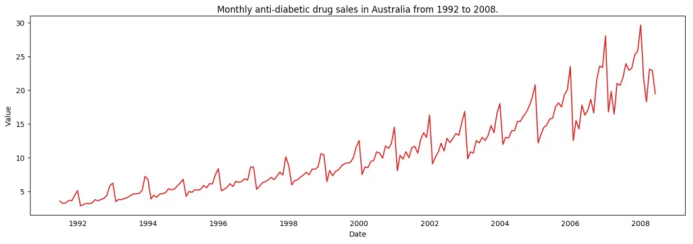时间序列可视化

因为所有的值都是正值，你可以在Y轴的两侧进行显示此值以强调增长。

# Import data
x = df['date'].values
y1 = df['value'].values

# Plot
fig, ax = plt.subplots(1, 1, figsize=(16,5), dpi= 120)
plt.fill_between(x, y1=y1, y2=-y1, alpha=0.5, linewidth=2, color='seagreen')
plt.ylim(-800, 800)
plt.title('Air Passengers (Two Side View)', fontsize=16)
plt.hlines(y=0, xmin=np.min(df.date), xmax=np.max(df.date), linewidth=.5)
plt.show()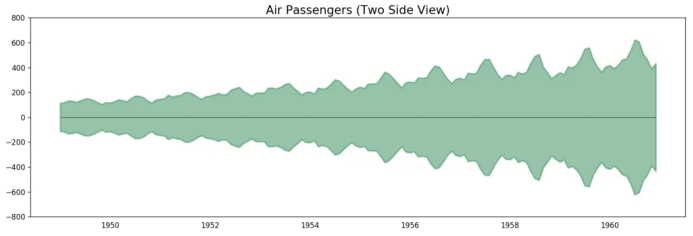航空乘客数据——两侧序列

因为这是一个月度时间序列，每年遵循特定的重复模式，你可以把每年作为一个单独的线画在同一张图上。这可以让你同时比较不同年份的模式。

4.1 时间序列的季节图

# Import Data
df.reset_index(inplace=True)

# Prepare data
df['year'] = [d.year for d in df.date]
df['month'] = [d.strftime('%b') for d in df.date]
years = df['year'].unique()

# Prep Colors
np.random.seed(100)
mycolors = np.random.choice(list(mpl.colors.XKCD_COLORS.keys()), len(years), replace=False)

# Draw Plot
plt.figure(figsize=(16,12), dpi= 80)
for i, y in enumerate(years):
if i > 0:
plt.plot('month', 'value', data=df.loc[df.year==y, :], color=mycolors[i], label=y)
plt.text(df.loc[df.year==y, :].shape-.9, df.loc[df.year==y, 'value'][-1:].values, y, fontsize=12, color=mycolors[i])

# Decoration
plt.gca().set(xlim=(-0.3, 11), ylim=(2, 30), ylabel='$Drug Sales$', xlabel='$Month$')
plt.yticks(fontsize=12, alpha=.7)
plt.title("Seasonal Plot of Drug Sales Time Series", fontsize=20)
plt.show()药品销售的季节图

每年二月会迎来药品销售的急速下降，而在三月会再度上升，接下来的4月又开始下降，以此类推。很明显，该模式在特定的某一年中重复，且年年如此。

然而，随着年份推移，药品销售整体增加。你可以很好地看到该趋势并且在年份箱线图当中看到它是怎样变化的。同样地，你也可以做一个月份箱线图来可视化月度分布情况。

4.2 月度（季节性）箱线图和年度（趋势）分布

你可以季节间隔将数据分组，并看看在给定的年份或月份当中值是如何分布的，以及随时间推移它们是如何比较的。

# Import Data
df.reset_index(inplace=True)

# Prepare data
df['year'] = [d.year for d in df.date]
df['month'] = [d.strftime('%b') for d in df.date]
years = df['year'].unique()

# Draw Plot
fig, axes = plt.subplots(1, 2, figsize=(20,7), dpi= 80)
sns.boxplot(x='year', y='value', data=df, ax=axes)
sns.boxplot(x='month', y='value', data=df.loc[~df.year.isin([1991, 2008]), :])

# Set Title
axes.set_title('Year-wise Box Plot\n(The Trend)', fontsize=18);
axes.set_title('Month-wise Box Plot\n(The Seasonality)', fontsize=18)
plt.show()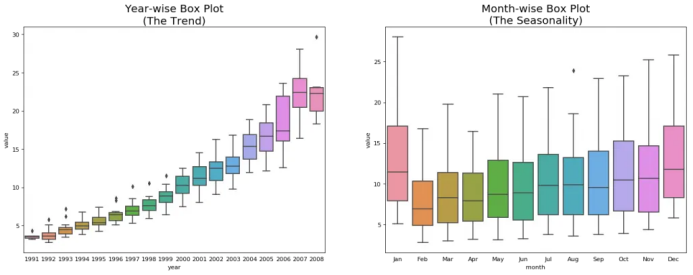年度和月度箱线图

箱线图将年度和月度分布变得很清晰。并且，在阅读箱线图当中，12月和1月明显有更高的药品销售量，可被归因于假期折扣季。

到目前为止，我们已经看到了识别模式的相似之处。现在怎样才能从通常模式当中找到离群值呢？

## 5. 时间序列的模式

任何时间序列都可以被分解为如下的部分：基线水平+趋势+季节性+误差

当在时间序列当中观测到增加或降低的斜率时，即可观测到相应的趋势。然而季节性只有在由于季节性因素导致不同的重复模式在规律性的间隔之间被观测到时才能发现。可能是由于当年的特定月份，特定月份的某一天、工作日或者甚至是当天某个时间。

然而，并不是所有时间序列必须有一个趋势和/或季节性。时间序列可能没有不同的趋势但是有一个季节性。反之亦然。

所以时间序列可以被看做是趋势、季节性和误差项的整合。

fig, axes = plt.subplots(1,3, figsize=(20,4), dpi=100)
pd.read_csv('https://raw.githubusercontent.com/selva86/datasets/master/guinearice.csv', parse_dates=['date'], index_col='date').plot(title='Trend Only', legend=False, ax=axes)

pd.read_csv('https://raw.githubusercontent.com/selva86/datasets/master/sunspotarea.csv', parse_dates=['date'], index_col='date').plot(title='Seasonality Only', legend=False, ax=axes)

pd.read_csv('https://raw.githubusercontent.com/selva86/datasets/master/AirPassengers.csv', parse_dates=['date'], index_col='date').plot(title='Trend and Seasonality', legend=False, ax=axes)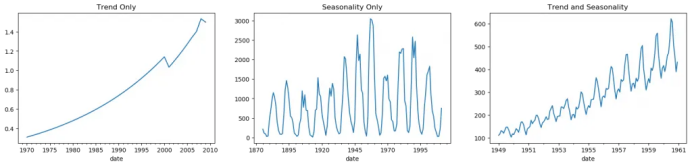时间序列中的模式

另一个需要考虑的方面是循环的行为。当序列当中上升和下降模式并不在固定的日历间隔出现时，就会出现循环的行为。需注意不要混淆循环的效应和季节的效应。

所以，怎样区分循环的和季节性的模式呢？

如果模式不是基于固定的日历频率，那它就是循环的。因为，循环效应不像季节性那样受到商业和其他社会经济因素的影响。

## 6. 时间序列的加法和乘法

基于趋势和季节性的本质，时间序列以加法或乘法的形式建模，其中序列里的每个观测值可被表达为成分的和或者积：

加法时间序列：值=基线水平+趋势+季节性+误差

乘法时间序列：值=基线水平*趋势*季节性*误差

## 7. 怎样分解时间序列的成分？

你可以通过将序列作基线水平，趋势，季节性指数和残差的加法或乘法组合来实现一个经典的时间序列分解。

statsmodels包里的seasonal_decompose使用起来非常方便。

from statsmodels.tsa.seasonal import seasonal_decompose
from dateutil.parser import parse

# Import Data

# Multiplicative Decomposition
result_mul = seasonal_decompose(df['value'], model='multiplicative', extrapolate_trend='freq')

# Plot
plt.rcParams.update({'figure.figsize': (10,10)})
result_mul.plot().suptitle('Multiplicative Decompose', fontsize=22)
plt.show()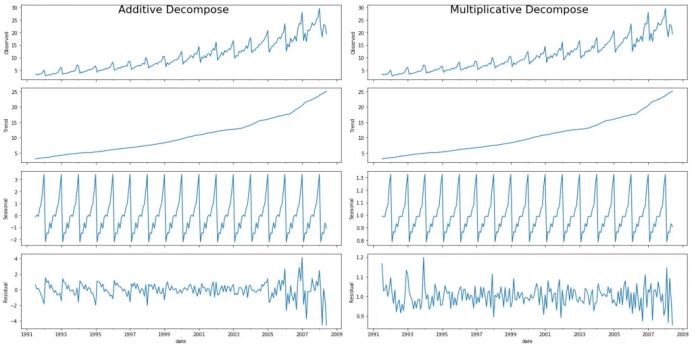加法和乘法分解

在序列开始时，设置extrapolate_trend='freq' 来注意趋势和残差中缺失的任何值。

如果你仔细看加法分解当中的残差，它有一些遗留模式。乘法分解看起来非常随意，这很好。所以理想状况下，乘法分解应该在这种特定的序列当中优先选择。

趋势，季节性和残差成分的数值输出被存储在result_mul 当中。让我们提取它们并导入数据框中。

# Extract the Components ----
# Actual Values = Product of (Seasonal * Trend * Resid)
df_reconstructed = pd.concat([result_mul.seasonal, result_mul.trend, result_mul.resid, result_mul.observed], axis=1)
df_reconstructed.columns = ['seas', 'trend', 'resid', 'actual_values']


如果你检查一下seas, trend 和 resid列的乘积，应该确实等于actual_values。

## 8. 平稳和非平稳时间序列

平稳性是时间序列的属性之一。平稳序列的值不是时间的函数。

也就是说，这种序列的统计属性例如均值，方差和自相关是随时间不变的常数。序列的自相关只是与前置值的相关，之后会详细介绍。

平稳时间序列也没有季节效应。

所以如何识别一个序列是否平稳呢？让我们通过实例来展示一下：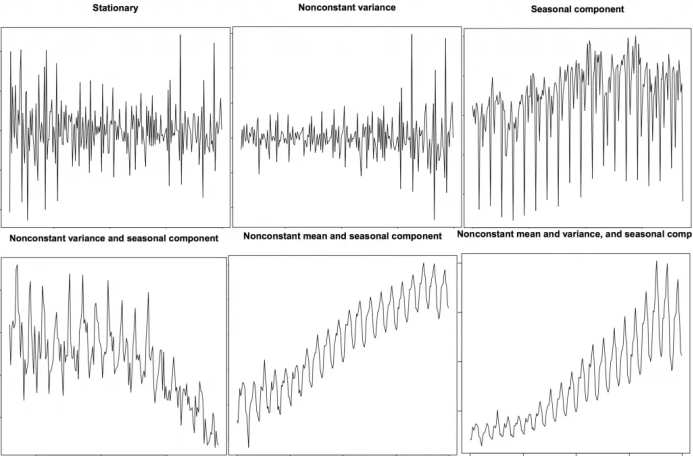平稳和非平稳时间序列

上图来自R语言的 TSTutorial。

所以为什么平稳序列是重要的呢？为什么我要提到它？

我将展开讲一下，但是要理解它只是有可能通过使用特定的转换方法实现任何时间序列的平稳化。大多数统计预测方法都用于平稳时间序列。预测的第一步通常是做一些转换将非平稳数据转化为平稳数据。

## 9. 如何获取平稳的时间序列？

你可以通过以下步骤实现序列的平稳化：

1. 差分序列（一次或多次）；

2. 对序列值进行log转换；

3. 对序列值去n次根式值；

4. 结合上述方法。

实现数据平稳化最常见也最方便的方法是对序列进行差分至少一次，直到它变得差不多平稳为止。

9.1 所以什么是差分？

如果Y_t是t时刻的Y值，那么第一次差分Y = Yt – Yt-1。在简化的格式当中，差分序列就是从当前值中减去下一个值。

如果第一次差分不能使数据平稳，你可以第二次差分，以此类推。

例如，考虑如下序列: [1, 5, 2, 12, 20]

一次差分: [5-1, 2-5, 12-2, 20-12] = [4, -3, 10, 8]

二次差分: [-3-4, -10-3, 8-10] = [-7, -13, -2]

## 9.2 为什么要在预测之前将非平稳数据平稳化？

预测平稳序列相对容易，预测也相对更可靠。

一个重要的原因是自回归预测模型必须是利用序列自身的滞后量作为预测变量的线性回归模型。

我们知道线性回归在预测变量（X变量）与其他变量不相关时效果最佳。所以序列平稳化也因为移除所有持续的自相关而解决了这个问题，因此使得模型中的预测变量（序列的滞后值）几乎独立。

现在我们已经建立了序列平稳化非常重要的概念，那怎样检验给定序列是否平稳化呢？

## 10. 怎样检验平稳性?

序列的平稳性可以通过之前我们提到的序列图看出来。

另外一种方法是将序列分成2或多个连续的部分，计算概要统计量例如均值，方差和自相关。如果统计量显著差异，序列可能不是平稳的。

尽管如此，你需要一个方法来从量化的角度判断一个给定序列是否平稳。可以通过‘Unit Root Tests单位根检验’来实现。这里有多种变式，但这些检验都是用来检测时间序列是否非平稳并且拥有一个单位根。

有多种单位根检验的具体应用：

2. 科维亚特夫斯基-菲利普斯-施密特-辛-KPSS检验（趋势平稳性）；

3. 菲利普斯 佩龙检验（PP Test）。

from statsmodels.tsa.stattools import adfuller, kpss

print(f'p-value: {result}')
for key, value in result.items():
print('Critial Values:')
print(f'   {key}, {value}')

# KPSS Test
result = kpss(df.value.values, regression='c')
print('\nKPSS Statistic: %f' % result)
print('p-value: %f' % result)
for key, value in result.items():
print('Critial Values:')
print(f'   {key}, {value}')
p-value: 1.0
Critial Values:
1%, -3.465620397124192
Critial Values:
5%, -2.8770397560752436
Critial Values:
10%, -2.5750324547306476

KPSS Statistic: 1.313675
p-value: 0.010000
Critial Values:
10%, 0.347
Critial Values:
5%, 0.463
Critial Values:
2.5%, 0.574
Critial Values:
1%, 0.739


## 11. 白噪音和平稳序列的差异是什么？

如平稳序列，白噪音也不是时间的函数，它的均值和方差并不随时间变化。但是它与平稳序列的差异在于，白噪音完全随机，均值为0。

无论怎样，在白噪音当中是没有特定模式的。如果你将FM广播的声音信号作为时间序列，你在频道之间的频段听到的空白声就是白噪音。

从数学上来看，均值为0的完全随机的数字序列是白噪音。

randvals = np.random.randn(1000)
pd.Series(randvals).plot(title='Random White Noise', color='k')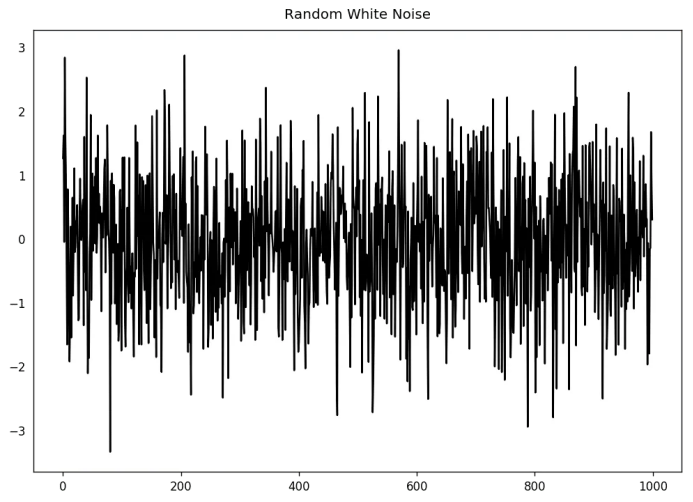随机白噪音

## 12. 怎样将时间序列去趋势化？

对时间序列去趋势就是从时间序列当中移除趋势成分。但是如何提取趋势呢？有以下几个方法。

1. 从时间序列当中减去最优拟合线。最佳拟合线可从以时间步长为预测变量获得的线性回归模型当中获得。对更复杂的模型，你可以使用模型中的二次项（x^2）；

2. 从我们之前提过的时间序列分解当中减掉趋势成分；

3. 减去均值；

4. 应用像Baxter-King过滤器(statsmodels.tsa.filters.bkfilter)或者Hodrick-Prescott 过滤器 (statsmodels.tsa.filters.hpfilter)来去除移动的平均趋势线或者循环成分。

让我们来用一下前两种方法。

# Using scipy: Subtract the line of best fit
from scipy import signal
detrended = signal.detrend(df.value.values)
plt.plot(detrended)
plt.title('Drug Sales detrended by subtracting the least squares fit', fontsize=16)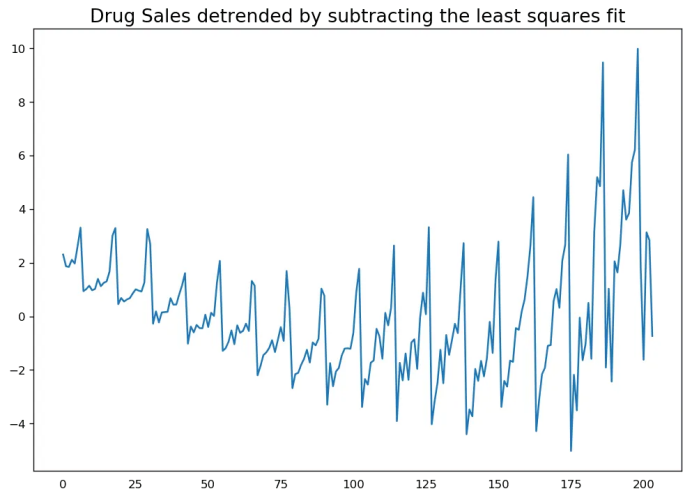通过减去最小二乘拟合来对时间序列去趋势化

# Using statmodels: Subtracting the Trend Component.
from statsmodels.tsa.seasonal import seasonal_decompose
result_mul = seasonal_decompose(df['value'], model='multiplicative', extrapolate_trend='freq')
detrended = df.value.values - result_mul.trend
plt.plot(detrended)
plt.title('Drug Sales detrended by subtracting the trend component', fontsize=16)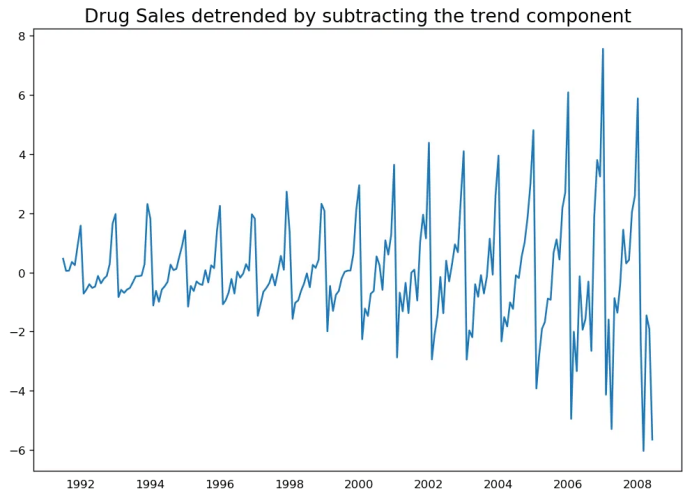通过减去趋势成分来去趋势化

## 13. 怎样对时间序列去季节化?

这里有多种方法对时间序列去季节化。以下就有几个：

1. 取一个以长度为季节窗口的移动平均线。这将在这个过程中使序列变得平滑；

2. 序列季节性差分（从当前值当中减去前一季节的值）；

3. 将序列值除以从STL分解当中获得的季节性指数。

如果除以季节性指数后仍没办法得到良好的结果，再试一下序列对数转换然后再做。你之后可以通过去指数恢复到原始尺度。

# Subtracting the Trend Component.

# Time Series Decomposition
result_mul = seasonal_decompose(df['value'], model='multiplicative', extrapolate_trend='freq')

# Deseasonalize
deseasonalized = df.value.values / result_mul.seasonal

# Plot
plt.plot(deseasonalized)
plt.title('Drug Sales Deseasonalized', fontsize=16)
plt.plot()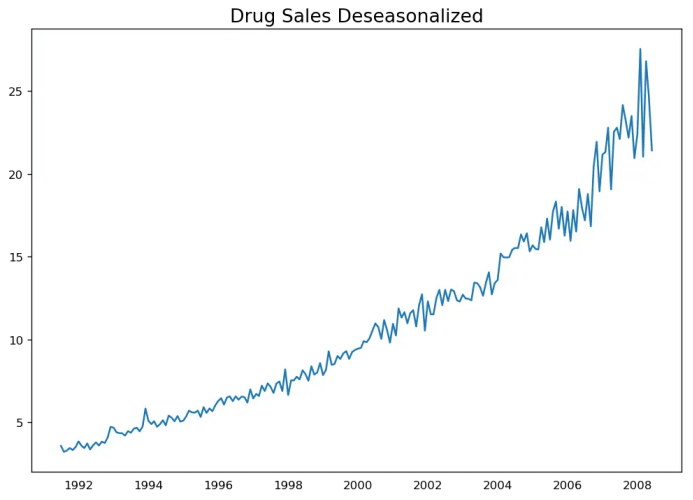时间序列去季节化

## 14. 怎样检验时间序列的季节性？

常见的方法是绘制序列并在固定的时间间隔内检查可重复的模式。所以，季节性的类型由钟表或日历决定：

1. 一天的每个小时；

2. 一月的每天；

3. 每周；

4. 每月；

5. 每年。

然而，如果你想要一个更权威的季节性检验，使用自回归函数（ACF）图。更多关于自回归的信息将在下一部分介绍。但是当强季节性模式出现时，ACF图通常揭示了在季节窗的倍数处明显的重复峰值。

例如，药品销售时间序列是每年都有重复模式的一个月度序列。所以，你可以看到第12,24和36条线等的峰值。

我必须警告你在现实世界的数据集当中，这样强的模式很难见到，并且有可能被各种噪音所扭曲，所以你需要一双仔细的眼睛来捕获这些模式。

from pandas.plotting import autocorrelation_plot

# Draw Plot
plt.rcParams.update({'figure.figsize':(9,5), 'figure.dpi':120})
autocorrelation_plot(df.value.tolist())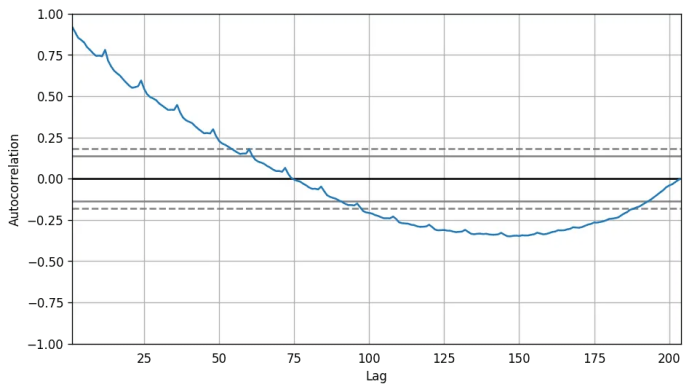自相关图

除此之外，如果你想做统计检验，CHT检验可以检验季节性差异是否对序列平稳化有必要。

## 15. 如何处理时间序列当中的缺失值？

有时，你的时间序列会有缺失日期/时间。那意味着，数据没有被捕获或者在那段时间内不可用。那些天的测量值有可能为0，你可以把那些时间段填充0。

其次，当处理时间序列时，你通常不应该用序列均值来替代缺失值，尤其是序列非平稳的时候，一个快捷粗略的处理方法来说你应该做的是向前填充之前的值。

然而，依赖于序列的本质，你想要在得出结论之前尝试多种方法。有效的缺失值处理方法有：

• 向后填充；

• 线性内插；

• 二次内插；

• 最邻近平均值；

• 对应季节的平均值。

为了衡量缺失值的表现，我在时间序列当中手动引入缺失值，使用上述方法处理并衡量处理值和真实值之间的均方误差。

# # Generate dataset
from scipy.interpolate import interp1d
from sklearn.metrics import mean_squared_error

fig, axes = plt.subplots(7, 1, sharex=True, figsize=(10, 12))
plt.rcParams.update({'xtick.bottom' : False})

## 1. Actual -------------------------------
df_orig.plot(title='Actual', ax=axes, label='Actual', color='red', style=".-")
df.plot(title='Actual', ax=axes, label='Actual', color='green', style=".-")
axes.legend(["Missing Data", "Available Data"])

## 2. Forward Fill --------------------------
df_ffill = df.ffill()
error = np.round(mean_squared_error(df_orig['value'], df_ffill['value']), 2)
df_ffill['value'].plot(title='Forward Fill (MSE: ' + str(error) +")", ax=axes, label='Forward Fill', style=".-")

## 3. Backward Fill -------------------------
df_bfill = df.bfill()
error = np.round(mean_squared_error(df_orig['value'], df_bfill['value']), 2)
df_bfill['value'].plot(title="Backward Fill (MSE: " + str(error) +")", ax=axes, label='Back Fill', color='firebrick', style=".-")

## 4. Linear Interpolation ------------------
df['rownum'] = np.arange(df.shape)
df_nona = df.dropna(subset = ['value'])
f = interp1d(df_nona['rownum'], df_nona['value'])
df['linear_fill'] = f(df['rownum'])
error = np.round(mean_squared_error(df_orig['value'], df['linear_fill']), 2)
df['linear_fill'].plot(title="Linear Fill (MSE: " + str(error) +")", ax=axes, label='Cubic Fill', color='brown', style=".-")

## 5. Cubic Interpolation --------------------
f2 = interp1d(df_nona['rownum'], df_nona['value'], kind='cubic')
df['cubic_fill'] = f2(df['rownum'])
error = np.round(mean_squared_error(df_orig['value'], df['cubic_fill']), 2)
df['cubic_fill'].plot(title="Cubic Fill (MSE: " + str(error) +")", ax=axes, label='Cubic Fill', color='red', style=".-")

# Interpolation References:
# https://docs.scipy.org/doc/scipy/reference/tutorial/interpolate.html
# https://docs.scipy.org/doc/scipy/reference/interpolate.html

## 6. Mean of 'n' Nearest Past Neighbors ------
def knn_mean(ts, n):
out = np.copy(ts)
for i, val in enumerate(ts):
if np.isnan(val):
n_by_2 = np.ceil(n/2)
lower = np.max([0, int(i-n_by_2)])
upper = np.min([len(ts)+1, int(i+n_by_2)])
ts_near = np.concatenate([ts[lower:i], ts[i:upper]])
out[i] = np.nanmean(ts_near)
return out

df['knn_mean'] = knn_mean(df.value.values, 8)
error = np.round(mean_squared_error(df_orig['value'], df['knn_mean']), 2)
df['knn_mean'].plot(title="KNN Mean (MSE: " + str(error) +")", ax=axes, label='KNN Mean', color='tomato', alpha=0.5, style=".-")

## 7. Seasonal Mean ----------------------------
def seasonal_mean(ts, n, lr=0.7):
"""
Compute the mean of corresponding seasonal periods
ts: 1D array-like of the time series
n: Seasonal window length of the time series
"""
out = np.copy(ts)
for i, val in enumerate(ts):
if np.isnan(val):
ts_seas = ts[i-1::-n]  # previous seasons only
if np.isnan(np.nanmean(ts_seas)):
ts_seas = np.concatenate([ts[i-1::-n], ts[i::n]])  # previous and forward
out[i] = np.nanmean(ts_seas) * lr
return out

df['seasonal_mean'] = seasonal_mean(df.value, n=12, lr=1.25)
error = np.round(mean_squared_error(df_orig['value'], df['seasonal_mean']), 2)
df['seasonal_mean'].plot(title="Seasonal Mean (MSE: " + str(error) +")", ax=axes, label='Seasonal Mean', color='blue', alpha=0.5, style=".-")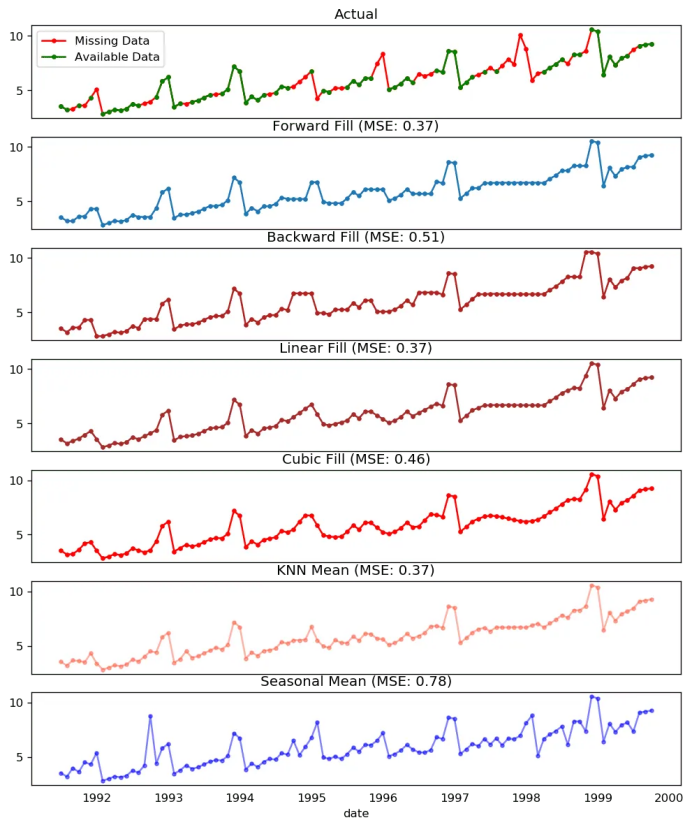缺失值处理

你也可以根据你想实现的精确程度考虑接下来的方法。

1. 如果你有解释变量，可以使用像随机森林或k-邻近算法的预测模型来预测它。

2. 如果你有足够多的过去观测值，可以预测缺失值。

3. 如果你有足够的未来观测值，回测缺失值。

4. 从之前的周期预测相对应的部分。

## 16. 什么是自相关和偏自相关函数？

自相关是序列和自己滞后量的简单相关。如果序列显著自相关，均值和序列之前的值（滞后量）可能对预测当前值有帮助。

偏自相关也会传递相似的信息但是它传递的是序列和它滞后量的纯粹相关，排除了其他中间滞后量对相关的贡献。

from statsmodels.tsa.stattools import acf, pacf
from statsmodels.graphics.tsaplots import plot_acf, plot_pacf

# Calculate ACF and PACF upto 50 lags
# acf_50 = acf(df.value, nlags=50)
# pacf_50 = pacf(df.value, nlags=50)

# Draw Plot
fig, axes = plt.subplots(1,2,figsize=(16,3), dpi= 100)
plot_acf(df.value.tolist(), lags=50, ax=axes)
plot_pacf(df.value.tolist(), lags=50, ax=axes)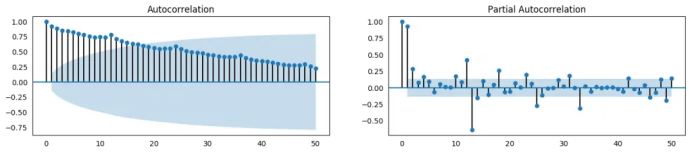自相关函数 和 偏自相关函数

## 17. 怎样计算偏自相关函数?

怎样计算偏自相关呢?

序列滞后量（k）的偏自相关是Y的自回归方程中滞后量的系数。Y的自回归方程就是Y及其滞后量作为预测项的线性回归。

For Example, if Y_t is the current series and Y_t-1 is the lag 1 of Y, then the partial autocorrelation of lag 3 (Y_t-3) is the coefficient $\alpha_3$ of Y_t-3 in the following equation:

例如，如果Y_t是当前的序列，Y_t-1是Y的滞后量1，那么滞后量3（Y_t-3）的偏自相关就是下面方程中Y_t-3的系数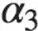: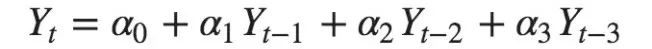自回归方程

## 18. 滞后图

滞后图是一个时间序列对其自身滞后量的散点图。它通常用于检查自相关。如果序列中存在如下所示的任何模式，则该序列是自相关的。如果没有这样的模式，这个序列很可能是随机的白噪声。

在下面太阳黑子面积时间序列的例子当中，随着n_lag增加，图越来越分散。

from pandas.plotting import lag_plot

# Import

# Plot
fig, axes = plt.subplots(1, 4, figsize=(10,3), sharex=True, sharey=True, dpi=100)
for i, ax in enumerate(axes.flatten()[:4]):
lag_plot(ss.value, lag=i+1, ax=ax, c='firebrick')
ax.set_title('Lag ' + str(i+1))

fig.suptitle('Lag Plots of Sun Spots Area \n(Points get wide and scattered with increasing lag -> lesser correlation)\n', y=1.15)

fig, axes = plt.subplots(1, 4, figsize=(10,3), sharex=True, sharey=True, dpi=100)
for i, ax in enumerate(axes.flatten()[:4]):
lag_plot(a10.value, lag=i+1, ax=ax, c='firebrick')
ax.set_title('Lag ' + str(i+1))

fig.suptitle('Lag Plots of Drug Sales', y=1.05)
plt.show()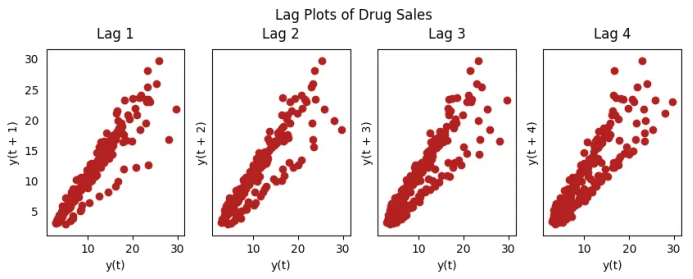药品销售的滞后图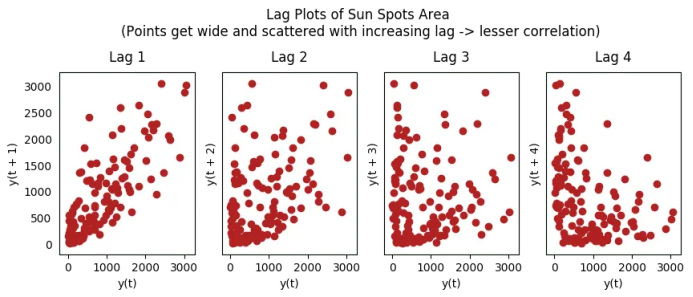太阳黑子的滞后图

## 19. 怎样估计时间序列的预测能力?

时间序列越有规律性和重复性的模式，越容易被预测。“近似熵”可用于量化时间序列波动的规律性和不可预测性。

近似熵越高，预测越难。另一个更好的选项是“样本熵”。

样本熵类似与近似熵，但是在估计小时间序列的复杂性上结果更一致。例如，较少样本点的随机时间序列 “近似熵”可能比一个更规律的时间序列更低，然而更长的时间序列可能会有一个更高的“近似熵”。

样本熵可以很好地处理这个问题。请看如下演示：

# https://en.wikipedia.org/wiki/Approximate_entropy
rand_small = np.random.randint(0, 100, size=36)
rand_big = np.random.randint(0, 100, size=136)

def ApEn(U, m, r):
"""Compute Aproximate entropy"""
def _maxdist(x_i, x_j):
return max([abs(ua - va) for ua, va in zip(x_i, x_j)])

def _phi(m):
x = [[U[j] for j in range(i, i + m - 1 + 1)] for i in range(N - m + 1)]
C = [len([1 for x_j in x if _maxdist(x_i, x_j) <= r]) / (N - m + 1.0) for x_i in x]
return (N - m + 1.0)**(-1) * sum(np.log(C))

N = len(U)
return abs(_phi(m+1) - _phi(m))

print(ApEn(ss.value, m=2, r=0.2*np.std(ss.value)))     # 0.651
print(ApEn(a10.value, m=2, r=0.2*np.std(a10.value)))   # 0.537
print(ApEn(rand_small, m=2, r=0.2*np.std(rand_small))) # 0.143
print(ApEn(rand_big, m=2, r=0.2*np.std(rand_big)))     # 0.716
0.6514704970333534
0.5374775224973489
0.0898376940798844
0.7369242960384561
# https://en.wikipedia.org/wiki/Sample_entropy
def SampEn(U, m, r):
"""Compute Sample entropy"""
def _maxdist(x_i, x_j):
return max([abs(ua - va) for ua, va in zip(x_i, x_j)])

def _phi(m):
x = [[U[j] for j in range(i, i + m - 1 + 1)] for i in range(N - m + 1)]
C = [len([1 for j in range(len(x)) if i != j and _maxdist(x[i], x[j]) <= r]) for i in range(len(x))]
return sum(C)

N = len(U)
return -np.log(_phi(m+1) / _phi(m))

print(SampEn(ss.value, m=2, r=0.2*np.std(ss.value)))      # 0.78
print(SampEn(a10.value, m=2, r=0.2*np.std(a10.value)))    # 0.41
print(SampEn(rand_small, m=2, r=0.2*np.std(rand_small)))  # 1.79
print(SampEn(rand_big, m=2, r=0.2*np.std(rand_big)))      # 2.42
0.7853311366380039
0.41887013457621214
inf
2.181224235989778

del sys.path


## 20. 为何要以及怎样对时间序列进行平滑处理?

时间序列平滑处理可能在以下场景有用：

• 在信号当中减小噪声的影响从而得到一个经过噪声滤波的序列近似。

• 平滑版的序列可用于解释原始序列本身的特征。

• 趋势更好地可视化。

怎样对序列平滑处理？让我们讨论一下以下方法：

1. 使用移动平均；

2. 做LOESS光滑（局部回归）；

3. 做LOWESS光滑（局部加权回归）。

移动均值就是定义宽度的滚动窗口的均值。但是你必须明智地选择窗口宽度，因为大范围窗口可能会造成序列过度平滑。例如，窗口大小等于季节持续时间时（例如：12为月度序列），将有效地抵消季节效应。

LOESS，局部回归的简写，适应于每个点邻近的多元回归。可通过statsmodels包使用，你可以使用frac参数确定被纳入拟合回归模型的邻近数据点的百分比来控制平滑度。

下载数据集: Elecequip.csv

from statsmodels.nonparametric.smoothers_lowess import lowess

# Import

# 1. Moving Average
df_ma = df_orig.value.rolling(3, center=True, closed='both').mean()

# 2. Loess Smoothing (5% and 15%)
df_loess_5 = pd.DataFrame(lowess(df_orig.value, np.arange(len(df_orig.value)), frac=0.05)[:, 1], index=df_orig.index, columns=['value'])
df_loess_15 = pd.DataFrame(lowess(df_orig.value, np.arange(len(df_orig.value)), frac=0.15)[:, 1], index=df_orig.index, columns=['value'])

# Plot
fig, axes = plt.subplots(4,1, figsize=(7, 7), sharex=True, dpi=120)
df_orig['value'].plot(ax=axes, color='k', title='Original Series')
df_loess_5['value'].plot(ax=axes, title='Loess Smoothed 5%')
df_loess_15['value'].plot(ax=axes, title='Loess Smoothed 15%')
df_ma.plot(ax=axes, title='Moving Average (3)')
fig.suptitle('How to Smoothen a Time Series', y=0.95, fontsize=14)
plt.show()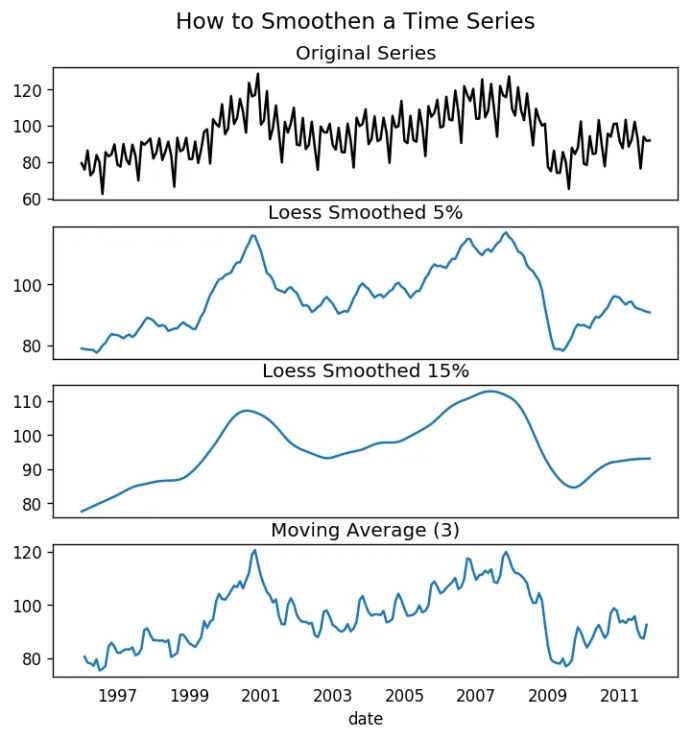平滑时间序列

## 21. 如何使用Granger因果检验得知是否一个时间序列有助于预测另一个序列？

Granger因果检验被用于检验是否一个时间序列可以预测另一个序列。Granger因果检验是如何工作的？

它基于如果X引起Y的变化，Y基于之前的Y值和之前的X值的预测效果要优于仅基于之前的Y值的预测效果。

所以需要了解Granger因果检验不能应用于Y的滞后量引起Y自身的变化的情况，而通常仅用于外源变量（不是Y的滞后量）。

它在statsmodel包中得到了很好的实现。它采纳2列数据的二维数组作为主要参数，被预测值是第一列，而预测变量（X）在第二列。

零假设检验：第二列的序列不能Granger预测第一列数据。如果p值小于显著性水平（0.05），你可以拒绝零假设并得出结论：X的滞后量确实有用。

第二个参数maxlag决定有多少Y的滞后量应该纳入检验当中。

from statsmodels.tsa.stattools import grangercausalitytests
df['month'] = df.date.dt.month
grangercausalitytests(df[['value', 'month']], maxlag=2)
Granger Causality
number of lags (no zero) 1
ssr based F test:         F=54.7797 , p=0.0000  , df_denom=200, df_num=1
ssr based chi2 test:   chi2=55.6014 , p=0.0000  , df=1
likelihood ratio test: chi2=49.1426 , p=0.0000  , df=1
parameter F test:         F=54.7797 , p=0.0000  , df_denom=200, df_num=1

Granger Causality
number of lags (no zero) 2
ssr based F test:         F=162.6989, p=0.0000  , df_denom=197, df_num=2
ssr based chi2 test:   chi2=333.6567, p=0.0000  , df=2
likelihood ratio test: chi2=196.9956, p=0.0000  , df=2
parameter F test:         F=162.6989, p=0.0000  , df_denom=197, df_num=2


在上述例子当中，实际上所有检验的p值只能无限接近于0。所以“月份”实际上可以用于预测航空乘客的数量。

## 22. 下一步是什么？

这就是我们现在要说的。我们从非常基础的内容开始，理解了时间序列不同特征。一旦分析完成之后，接下来的一步是预测。

原文标题：

Time Series Analysis in Python – A Comprehensive Guide with Examples

原文链接：

https://www.machinelearningplus.com/time-series/time-series-analysis-python/

编辑：黄继彦干货学习，三连

展开全文python 机器学习 深度学习
• 当我们有一个新的时间序列数据时，怎么判断它是否是平稳的呢？时间序列平稳性检验方法，可分为三类：图形分析方法简单统计方法假设检验方法一、图形分析方法图形分析方法是一种最基本、最简单直接的方法...算法 python 机器学习
• 简单来说，时间序列的回归分析需要我们分析历史数据，找到历史数据演化中的特征与模式，其主要分为线性回归分析和非线性回归分析两种类型。 01 模型构建与验证 回归分析多采用机器学习方法，我们首先需要明确机器...神经网络 大数据 算法 python 机器学习
• 这里与时间序列的预测有一点相似，不同的是，时间序列预测中我们看不到所要预测点后面的数据，而时序补缺中，我们可以分析缺失点前后的数据，从而更精准的对缺失数据进行填充。 数据填充基本分为统计方式填充与机器...算法 大数据 python 机器学习 人工智能
• ## 使用 LSTM 进行多变量时间序列预测

万次阅读 多人点赞 2022-01-11 10:13:30
使用 LSTM 进行端到端时间序列预测的完整代码和详细解释。 在本文中我们将使用深度学习方法 (LSTM) 执行多元时间序列预测。 我们先来了解两个主题—— 什么是时间序列分析？ 什么是 LSTM？ 时间序列分析：时间序列...lstm 深度学习 神经网络 机器学习
• 时间序列在生产和科学研究中，对某一个或者一组变量 进行观察测量，将在一系列时刻所得到的离散数字组成的序列集合，称之为时间序列。pandas生成时间序列过滤数据重采样插值滑窗数据平稳性与差...人工智能 python 机器学习 深度学习
• 时间序列的平稳性、随机性检验 在拿到时间序列数据后，首先要对数据的随机性和平稳性进行检测， 这两个检测是时间序列预测的重要部分。根据不同检测结果需要采取不同的分析方法。 为什么时间序列要求平稳性呢？平稳...python
• 最近一直在接触时间序列，所以打算写一些有关时间序列的文章，预测部分会从规则开始、到传统模型、到机器学习、再到深度学习，此外也会介绍一些时间序列的基本概念，包括自相关、平稳性、滞后性、季节...机器学习 人工智能 python 深度学习
• 卷积网络具有深度，可并行等多种特性，这种技术是否可以应用于解单维度的时间序列问题呢？本文介绍一种最近提出的新技术：时间卷积神经网络 (Temporal Convolutional Network，TCN)，由Lea等人于2016年首次提出，...卷积 神经网络 python 机器学习
• 1. 时间序列规则 1.1 什么是时间序列规则 对于赛题/业务的规则之前已经描述了它的重要性和应用，在此不再赘述。这章主要了解时间序列及其规则，和周期的应用。 1.1.1 时间序列 时间序列（或称动态数列）：指将同一...
• 【AI蜗牛车出品】手把手AI项目、时空序列、时间序列、白话机器学习、pytorch修炼 公众号：AI蜗牛车 保持谦逊、保持自律、保持进步 个人微信 备注：昵称+学校/公司+方向 如果没有备注不拉群！ 拉你进AI蜗牛车交流群机器学习 人工智能 python 数据挖掘
• 大多数现代开源AutoML框架并没有广泛地涵盖时间序列预测任务。本文中我们将深入地研究AutoML框架之一FEDOT，它可以自动化时间序列预测的机器学习管道设计。因此，我们将通过时间序列预测的现实世界任务详细解释FEDOT...机器学习 automl 数据挖掘
• ## LSTM多变量时间序列预测

千次阅读 多人点赞 2021-03-27 20:40:42LSTM keras
• ## 时间序列预测方法最全总结！

万次阅读 多人点赞 2021-03-12 00:15:38
时间序列预测就是利用过去一段时间的数据来预测未来一段时间内的信息，包括连续型预测（数值预测，范围估计）与离散型预测（事件预测）等，具有非常高的商业价值。需要明确一点的是，与回归分析预测模型...卷积 神经网络 算法 python 机器学习
• 本篇文章将总结时间序列预测方法，并将所有方法分类介绍并提供相应的python代码示例，以下是本文将要介绍的方法列表： 1、使用平滑技术进行时间序列预测 指数平滑 Holt-Winters 法 2、单变量时间序列预测 自回归 ...python 机器学习 人工智能 自回归
• ## 时间序列聚类

千次阅读 2020-12-28 21:50:38
时间序列的聚类在工业生产生活中非常常见，大到工业运维中面对海量KPI曲线的隐含关联关系的挖掘，小到股票收益曲线中的增长模式归类，都要用到时序聚类的方法帮助我们发现数据样本中一些隐含的、深层的信息。机器学习 数据挖掘 算法 人工智能 聚类
• 我们通过讨论时间序列 Transformer 可能的未来方向来结束这项工作，包括： 时间序列 Transformer 的归纳偏差 时间序列的 Transformers 和 GNN 时间序列的预训练 Transformer 时间序列的带有 NAS 的 Transformer 2 ...异常检测...